A recent Twitter thread about a counterfeit analog multiplier chip attracted my attention since I'm interested in both counterfeit integrated circuits and how analog computers multiply. In the thread, John McMaster decapped a suspicious AD633 analog multiplier chip and found an entirely different Rockwell RC4200 die inside. Why would someone do this? Probably because the RC4200 (1978) currently sells for about 85 cents, while the more modern laser-trimmed1 AD633 (1989) sells for about $7.2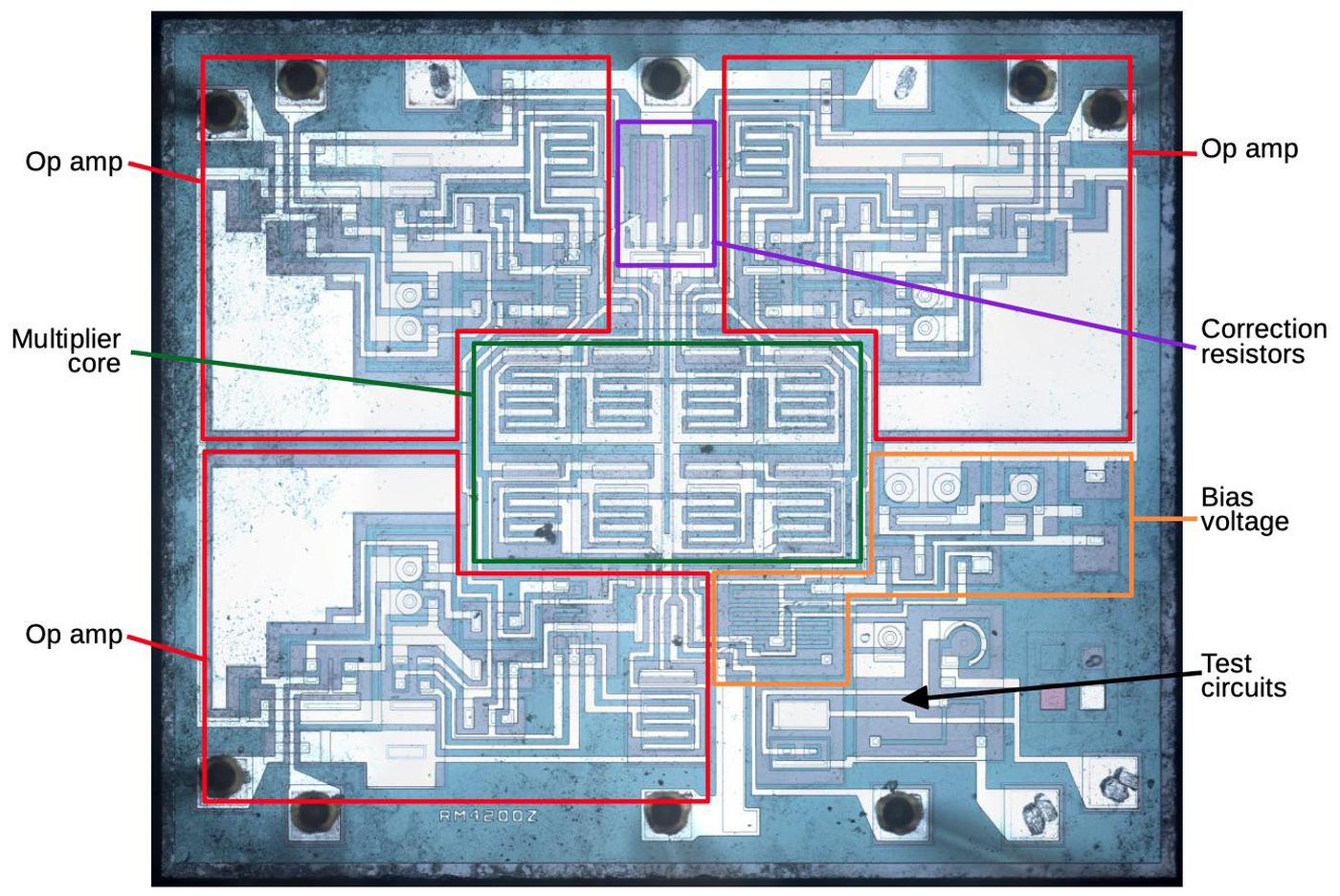Die of the RC4200 analog multiplier with functional blocks labeled. Die photo courtesy of John McMaster. Analog multiplication Analog multiplication has many uses such as mixers, modulators, and phase detectors, but analog computers are how I encountered analog multiplication. A typical analog computer uses voltages to represent values and is wired up through a plugboard to solve a particular equation. Adding or subtracting two values is easy with an op amp, as is multiplying by a constant. Integration seems like it would be difficult, but it's almost trivial with a capacitor; analog computers excelled at solving differential equations. Multiplying two values, however, was surprisingly difficult; multiplication techniques were slow, inaccurate, noisy, or expensive. One accurate but slow multiplier used the Rube-Goldberg configuration of servo motors turning potentiometers.3 A 1969 multiplier circuit uses a light bulb and photocells. A fast and accurate approach was the "parabolic multiplier", built from numerous expensive high-precision resistors.4 The approach I'll discuss is to multiply by adding the logarithms and taking the exponential. Inconveniently, this approach magnifies even small differences between the transistors. It is also very sensitive to temperature. As a result, this approach was simple but inaccurate.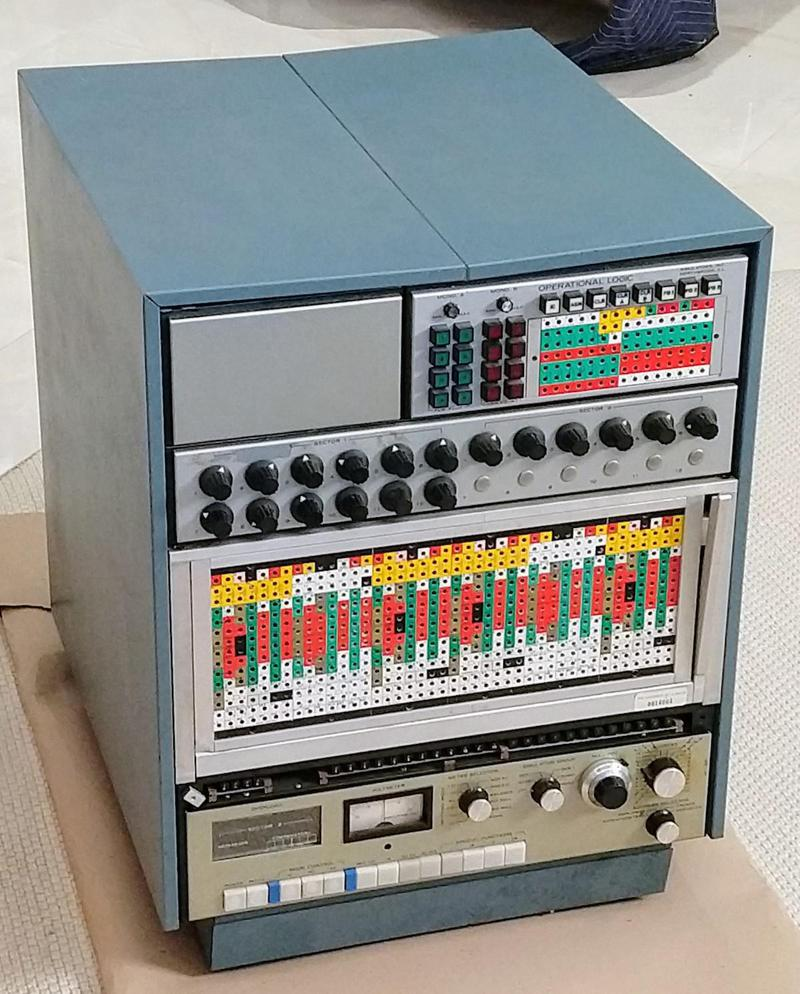The Model 240 analog computer from Simulators, Inc. includes analog multipliers using the parabolic multiplier approach. However, the development of analog integrated circuits created new opportunities for analog multiplication circuits. In particular, since the transistors in an integrated circuit were created together, they have nearly-identical properties. And the components on a tiny silicon die are all at nearly the same temperature.5 The first analog multiplier integrated circuit I could find is a television demodulator from 1967. The Gilbert cell technique was introduced by Barrie Gilbert in 1968 and is used in most analog multipliers today.6 The AD530 was introduced around 1970, and became an industry standard, but required external adjustments for accuracy. Laser-trimming the resistors inside the integrated circuit during manufacturing greatly improved the accuracy, an approach used in the AD633, the integrated circuit that was counterfeited. Before explaining the circuitry of the RC4200 (the multiplier inside the counterfeit chip), I'll discuss the components that it is constructed from, and how they appear in an integrated circuit. This will help you recognize these structures in the die photo. Transistors Transistors are the key components in a chip. The photo below shows an NPN transistor in the RC4200 as it appears on the chip. The different blue colors are regions of silicon that have been doped differently, forming N and P regions. The white lines are the metal layer of the chip on top of the silicon—these form the wires connecting to the emitter (E), base (B), and collector (C).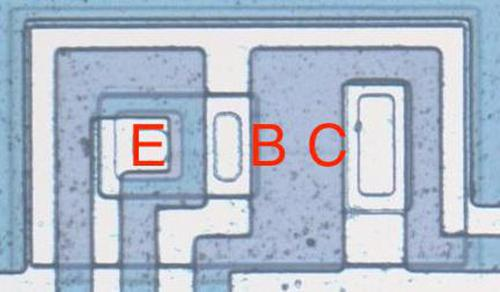An NPN transistor on the RC4200 die. The emitter is embedded in the base, with the collector underneath. You might expect PNP transistors to be similar to NPN transistors, just swapping the roles of N and P silicon. But for a variety of reasons, PNP transistors have an entirely different construction. They consist of a circular emitter (P), surrounded by a ring-shaped base (N), which is surrounded by the collector (P). This forms a P-N-P sandwich horizontally (laterally), unlike the vertical structure of the NPN transistors. The diagram below shows one of the PNP transistors in the RC4200.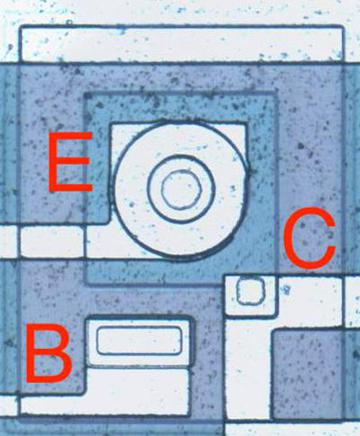A PNP transistor has a circular structure. The input and output transistors in the RC4200 are larger than the other transistors and have a different structure to support higher currents. The photo below shows one of the output transistors. Note the multiple interdigitated "fingers" of the emitter and base.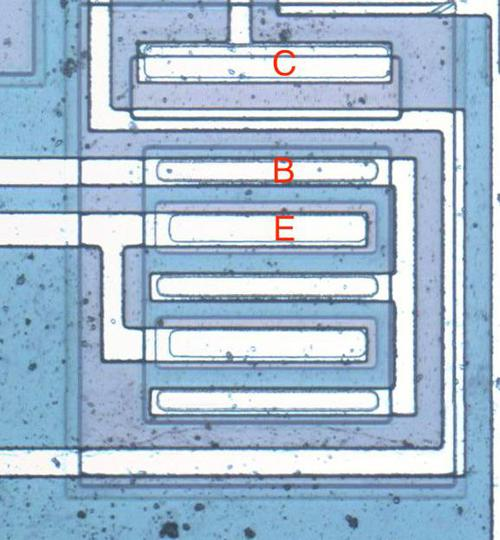A larger output transistor with parallel emitters and bases. Capacitors Capacitors are important in op amps to provide stability. A capacitor can be built in an integrated circuit as a large metal plate separated from the silicon by an insulating oxide layer. The main drawback of capacitors on ICs is they are physically very large. The 15pF capacitors in the RC4200 have a very small capacitance but take up a large fraction of the die area. In the photo below, the red arrows indicate the connection to the capacitor's metal layer and to the capacitor's underlying silicon layer.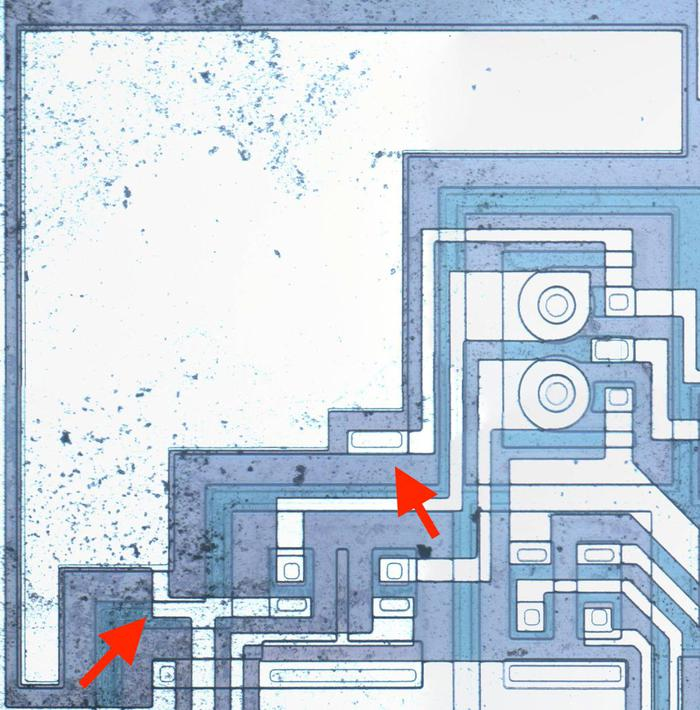The large metal area on the upper left is a capacitor. Resistors Resistors are a key component of analog chips. Unfortunately, resistors in ICs are very inaccurate; the resistances can vary by 50% from chip to chip. The photo below shows four resistors, formed using different techniques. The first resistor is the zig-zagging blue region on the left. It is formed from a strip of P silicon, with metal wiring (white) attached on the left and right. Its resistance is 3320 Ω. The resistor in the upper right is much shorter, so it is only 511Ω (long, narrow resistors have higher resistance than short, wide resistors). The remaining resistors are 20KΩ despite their small size because they are "pinch resistors". In the pinch resistor, the square layer of brownish N silicon on top makes the conductive region much thinner (i.e. pinches it). This allows a much higher resistance for a given size. (Otherwise, a 20 KΩ resistor would be 6 times as long as the first resistor, taking up excessive space.) The tradeoff is the pinch resistor is much less accurate.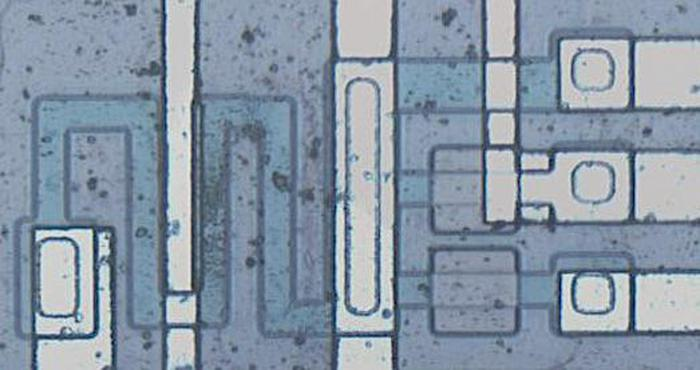Four resistors, one on the left and three on the right. Multiplying with logs and exponentials This integrated circuit multiplies using the log-antilog technique. The idea is that if you take the log of two numbers, add the logs together, and then take the antilog (i.e. exponential), you get the product of the two numbers. Conveniently, transistors have a logarithmic / exponential characteristic: the current through the transistor is an exponential of the voltage on the base. Specifically, if VBE is the voltage between the transistor's base and emitter, the current through the collector (IC) is an exponential of that voltage, as shown in the graph below. The analog multiplier takes advantage of this property.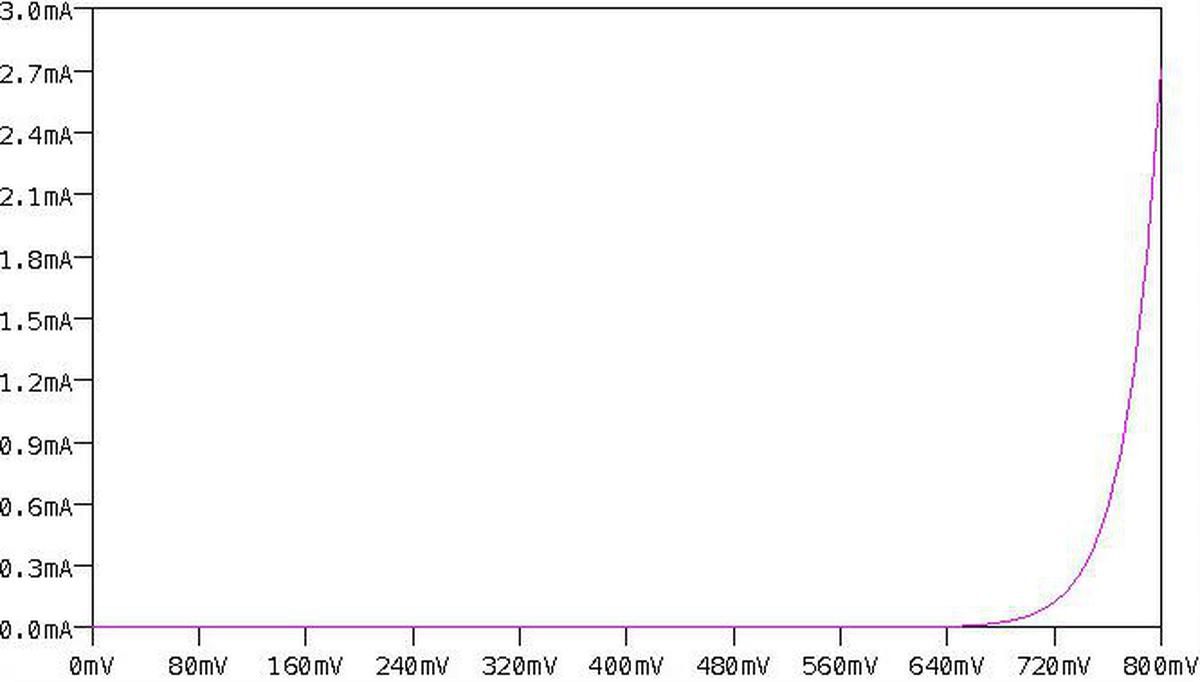Ic vs Vbe curve for a transistor, showing the exponential relationship. Generated by LTspice. The main complication with this approach is that the curve above is very sensitive to the temperature and to the manufacturing characteristics of the transistor. Because the curve is exponential, even a small shift in the curve will radically change the current. This was a serious difficulty when building a multiplier from discrete transistors, since the properties varied from transistor to transistor. To stabilize the temperature, some multipliers used a temperature-controlled oven. However, using an integrated circuit mostly solved these problems. The transistors in an integrated circuit are well-matched since they were built from the same piece of silicon under the same conditions. And the transistors in an integrated circuit die will be at almost the same temperature. Thus, integrated circuits made transistor-log circuits much more practical. The diagram below shows the structure of the RC4200 multiplier chip. The user provides three current inputs (I1, I2, and I4) and the chip computes the output current I3, where I3 = I1×I2÷I4. (The use of current inputs and outputs is a bit inconvenient compared to other multipliers, such as the AD633, that use voltages.)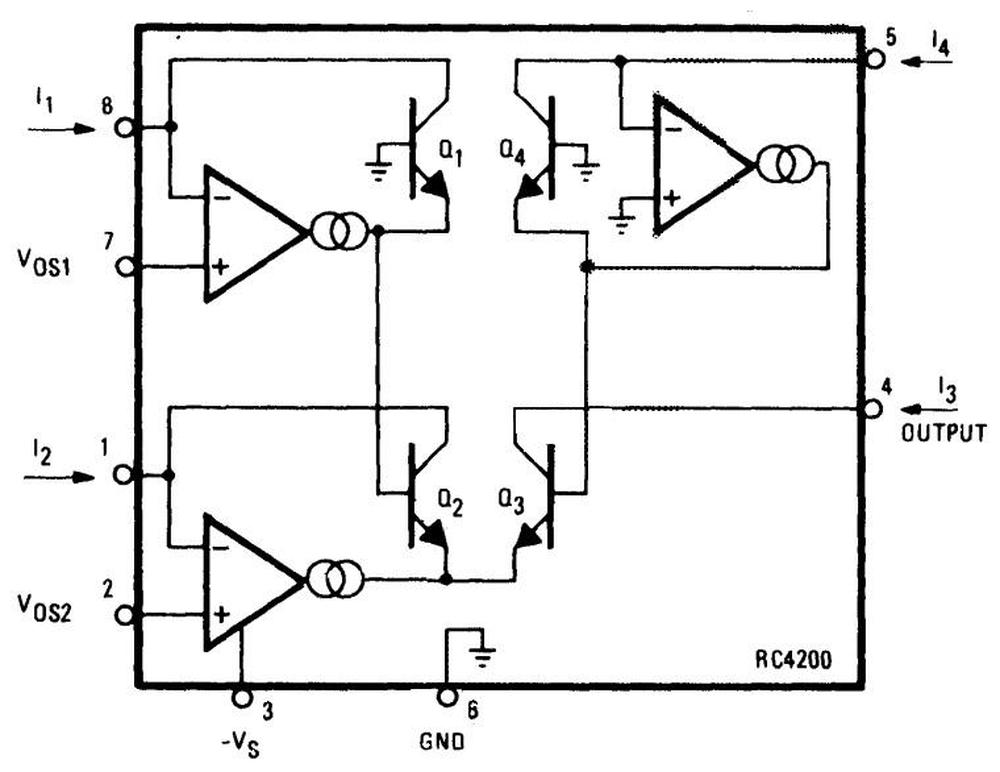Structure of the RC4200 multiplier, from the datasheet. Note that the supply voltage (pin 3) is negative. VOS1 and VOS2 are offset adjustment pins to improve accuracy. The four transistors in the middle of the diagram are the multiplier core, the key to the IC's operation. The transistors are configured so their base-emitter voltages sum: VBE3 = VBE1+VBE2-VBE4. Because the transistor current is related exponentially to the voltage, the result is that I3 = I1×I2÷I4. In more detail, first note that the voltages VBE1 through VBE4 control the collector currents IC1 through IC4 through the transistors (below). The op amps adjust the base-emitter voltages so the input currents match the transistor currents, i.e. I1 = IC1 and so forth. (This is accomplished by op amp feedback.) Now, if you go through the loop of base-emitter voltages starting at the base of Q1 and ending at the base of Q4 (red arrows), you find that VBE1+VBE2-VBE3-VBE4 = 0. (The voltages must sum to zero since you start at ground and end at ground.7) Now, because IC is related to exp(VBE), taking the exponential of the equation yields IC1×IC2÷IC3÷IC4 = 1. (Details in footnote8.)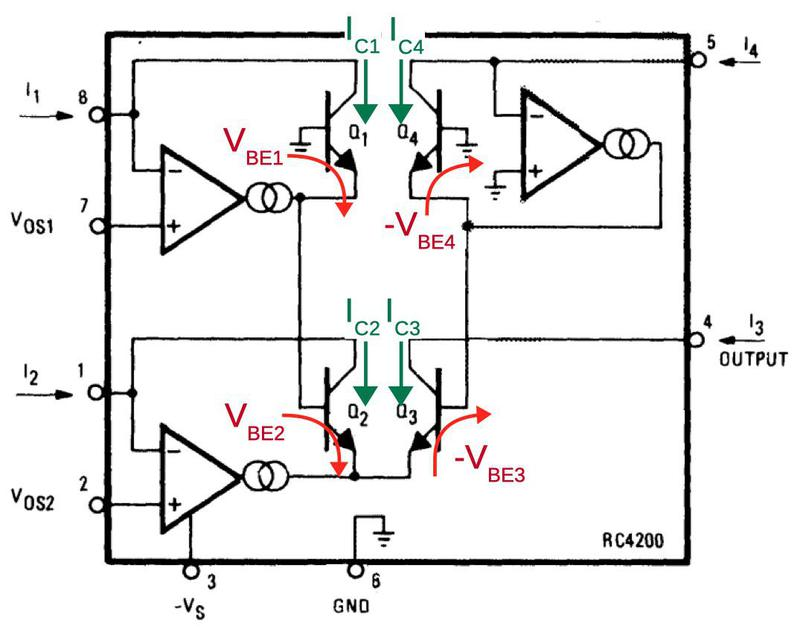Traveling around the loop indicated by the arrows, the voltages must sum to 0. Next, I'll explain how the VBE voltages are generated. Each current input has an op amp associated with it that produces the "correct" VBE voltage for the current using a feedback loop9 For example, suppose IC is too low so not all the input current flows through the transistor. The excess current will raise the voltage on the op amp's negative input, causing it to reduce its output voltage and thus the transistor's emitter voltage. This raises VBE (since the base will now be higher compared to the emitter), causing more collector current to flow through the transistor. Similarly, if too much current is flowing through the transistor, the op amp's input will be pulled lower, reducing VBE. Thus, the feedback loop causes the op amp to find the exact VBE for the current input.10 Correcting for emitter resistance The above circuit works reasonably well, but there's a complication: the transistors have a small emitter resistance R. The voltage drop across this resistance will increase VBE by ICR, disturbing the nice exponential behavior. This creates a nonlinearity that reduces the accuracy of the result. The datasheet says that "Raytheon has developed a unique and proprietary means of inherently compensating for this undesired term." They don't explain this further, but by studying the die I have figured out how it works. In the compensation circuit, each of the four multiplier transistors is paired with an identical "mirror" transistor with the corresponding emitters and corresponding bases connected, as shown below. These connections give the paired transistors the same base and emitter voltages, so they have the same collector currents. In other words, they form a current mirror. The mirrored currents are fed into special correction resistors that match the undesired emitter resistance, 0.1 Ω according to the datasheet.11 The voltage across the correction resistors will be the same as the excess voltage that needs to be compensated (since the resistance and current are the same). The final step is the correction resistors are connected to the base of the multiplication transistors, replacing the connection to ground. This will shrink VBE by the amount it was erroneously increased, fixing the computation.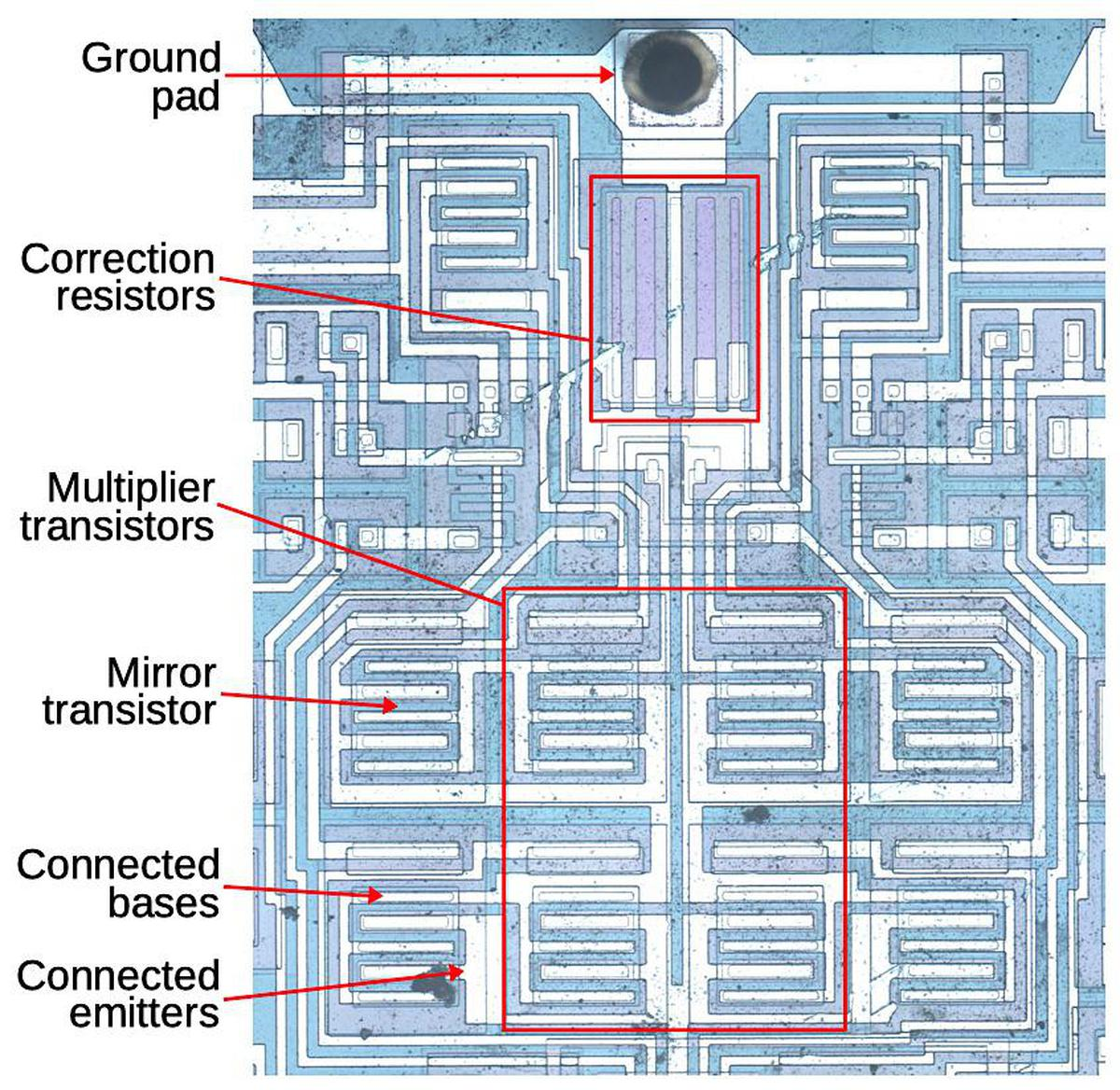The main multiplier consists of four transistors. Each transistor has a mirror transistor generating the same current, used to correct for emitter resistance. Why are there two correction resistors? Recall that the multiplier has two transistors adding and two transistors subtracting (i.e. VBE1+VBE2-VBE3-VBE4 = 0). To handle this, the correction circuit is split in two. The left half sums IC1 and IC2 and applies this current to a correction resistor on the Q3/Q4 side, while the right half sums IC3 and IC4 and applies this to a correction resistor on the Q1/Q2 side. The addition and subtraction work out to yield the desired net correction. Schematic The schematic below shows the complete circuitry of the RC4200; I've highlighted the main functional blocks. (Inconveniently, I didn't find this schematic until after I'd traced out the circuitry from the die photo.) The multiplier core and the correction resistors were discussed above The op amps circuits are fairly similar to the 741 op amp, which I've written about. They lack the output stage of typical op amps; the output transistor (Q112/Q212/Q412) corresponds to the intermediate gain state in a typical op amp. The bias circuit (orange, lower right) provides a fixed bias voltage for the op amps.12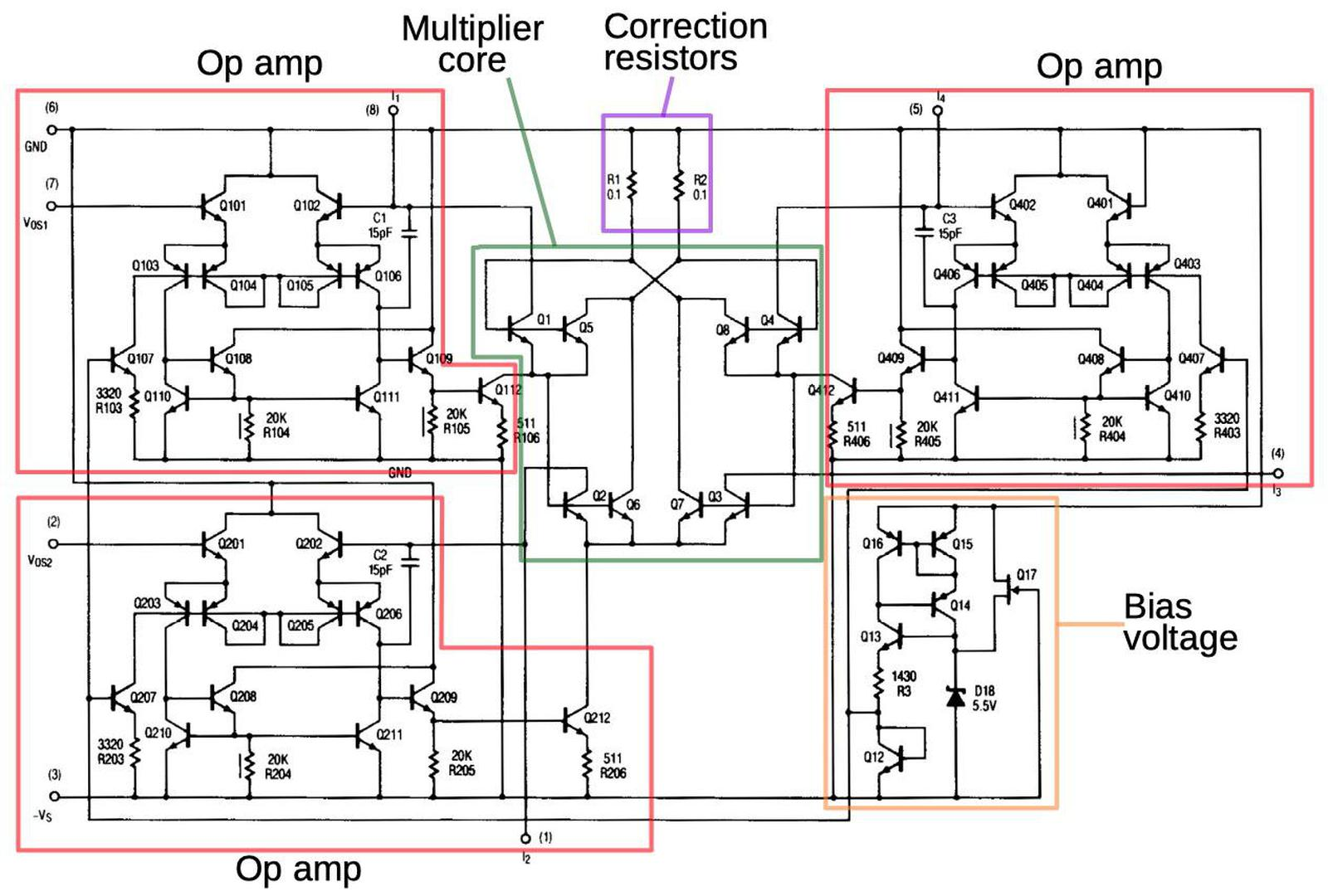Schematic from the datasheet, with main functional groups labeled. Conclusion Before integrated circuits, analog multiplication was difficult to implement. However, integrated circuits made it easy to create matched transistors, leading to fast, inexpensive analog multiplication integrated circuits. Unfortunately, analog multiplier integrated circuits were introduced just as analog computers were dying out, killed by inexpensive digital microprocessors, so analog computing missed most of the benefit of these chips. While most analog multipliers use a circuit called the Gilbert cell, the Raytheon RC4200 analog multiplier uses a different technique to multiply and divide values represented by currents. Although, it includes a special error compensation circuit to improve its accuracy, it is obsolete compared to accurate, laser-trimmed multipliers. Now, counterfeiters re-label RC4200 chips and sell them as the more-expensive AD633 multiplier.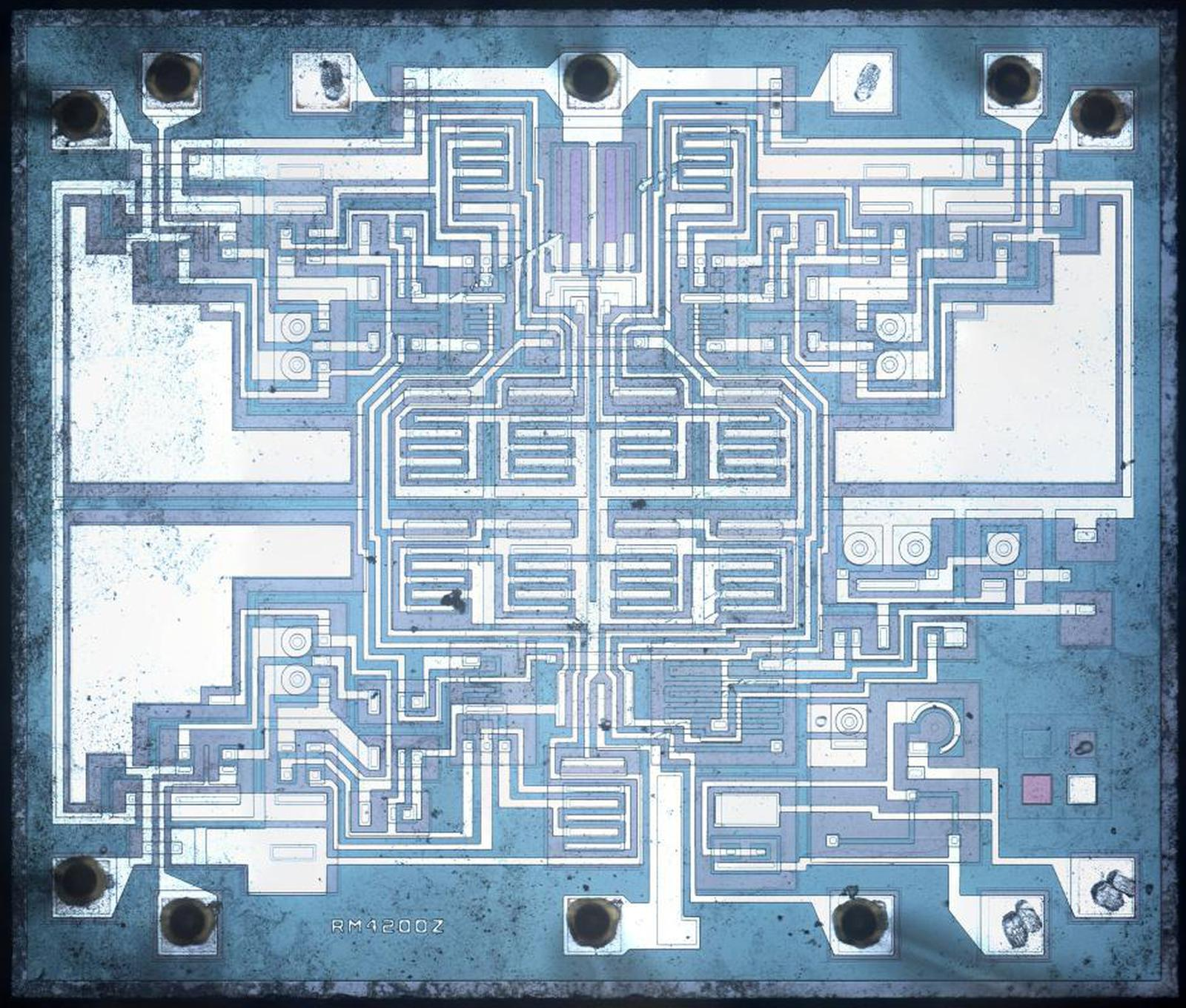Die photo of the RC4200, courtesy of John McMaster. I announce my latest blog posts on Twitter, so follow me at kenshirriff for updates. I also have an RSS feed. Thank you to John McMaster for the die photos used in this blog post; the photos are here. Notes and references 1. One reason that the AD633 multiplier is so expensive is that the resistors on the die are laser-trimmed resistors for accuracy. To get an accurate result, an analog multiplier requires exactly-tuned resistances. The older RC4200 requires adjustable external resistors, which is much less convenient. 2. I'm a bit puzzled by this counterfeit chip. Sometimes people will label a cheap op amp as an expensive op amp, as explained by Zeptobars. At first glance, that's what's going on here: a cheap multiplier repackaged as an expensive one. However, the two multipilers are so different that I can't imagine one working at all in place of the other. Specifically, the AD633 takes differential voltage inputs and outputs two currents (a differential current), and it computes A×B+C. The RC4200, on the other hand, takes current inputs and outputs a single current, and it computes A×B÷C. 3. An example of a servo multiplier is the Solartron Servo Multiplier from the late 1950s. This 17-pound unit contained a potentiometer controlled by a servo motor, allowing it to multiply numbers represented by +/- 100 volts. It's surprisingly fast considering its mechanical operation, responding in under 30 milliseconds. Power consumption was high: 70 watts, cooled by a fan. (In comparison, the RC4200 chip uses 40 milliwatts of power.) This photo shows the Solartron TJ961 Servo Resolver. This implements multiplication as well as sine/cosine computation. Photo from manual via Analog Museum. 4. The 1969 analog computer I'm restoring uses a parabolic multiplier, a technique used for high-accuracy multiplication. The idea is that to compute A×B, you compute ((A+B)^2 - (A-B)^2)/4, which has the same value. That equation looks much more complex than the original product, but is easier to implement on an analog computer because op amps can perform the sums, subtraction, and division by four. Squaring is easier than multiplication because it is a function of a single variable, so it can be implemented by an "arbitrary function generator".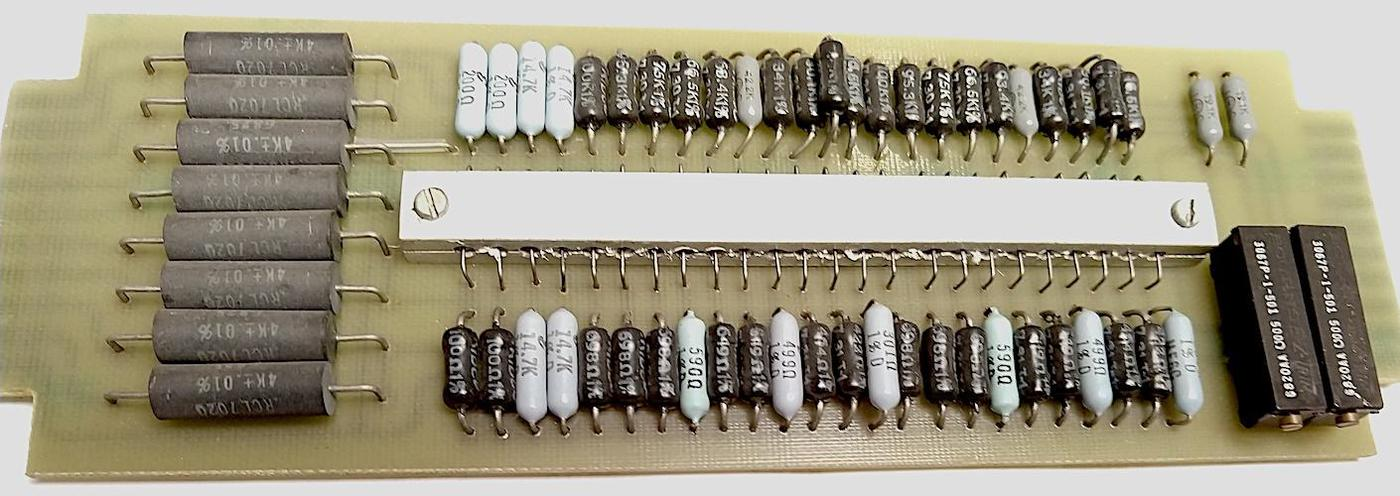Parabolic multiplier circuit board from a Simulators, Inc. 2400 analog computer. The photo above shows a function board from an analog computer that computes the square, i.e. a parabola. The board approximates the function by multiple piecewise-linear segments, each defined by resistors. (Note the extremely accurate 0.01% resistors on the left.) The metal block in the center holds diodes, temperature-balanced by the metal. Each diode is biased to turn on at a particular voltage; the diodes act as switches, selecting the appropriate resistors for each linear segment. Note the large amount of precision hardware required for multiplication; a single product requires two of these parabolic function boards as well as multiple op amps. 5. To minimize the effect of temperature on the integrated circuit, the critical multiplier transistors are placed close together in the center of the chip. If there is a thermal gradient across the chip, this will minimize the temperature difference between the transistors. (Compared to putting the transistors in the corners, for instance.) To reduce temperature gradients even more, the datasheet specifies a "thermal symmetry line". Putting a temperature source on this line ensures that the hotter transistors will tend to cancel each other out. The datasheet shows the IC's thermal symmetry line. 6. Barrie Gilbert, inventor of the Gilbert cell, has a video explaining translinear circuit, circuits based on the exponential current-voltage relationship of a bipolar transistor. This video explains translinear analog multipliers in detail, discussing two approaches> The first approach, used by the RC4200, is the "log-antilog" approach, where op-amps force and sense the collector currents. The second, used in the AD633 and many other multipliers, is the "integrated" approach, built from voltage-to-current conversion, a differential current-mode core, and current-to-voltage conversion. 7. I should mention that the chip uses a -15 V supply, so ground is the highest voltage and the other internal voltages are all negative. Just a warning since this makes things confusing and backward compared to circuits where ground is the low voltage. 8. The relationship between the base voltage and the collector current is given by the Ebers-Moll model. This equation (below) is filled with interesting constants: α: a gain factor (almost 1), k: the Boltzmann constant, IS: the saturation current (extremely small, order of 10-15 A), T: the absolute temperature, q: the charge on the electron. (The temperature in the exponential term reflects the importance of temperature stability for the multiplier.)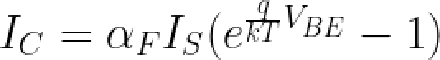Substituting the thermal voltage VT (about 26 mV) for kT/q, making some minor approximations, and taking the log yields: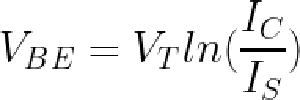Substituting that into the multiplier's VBE loop equation yields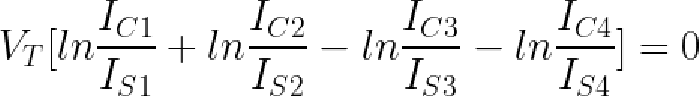Taking the exponential and assuming the transistors all have the same temperature and saturation current yields the desired equation relating the four currents: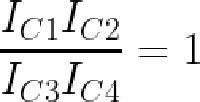This equation shows how the four currents are related by multiplication and division. See the datasheet for more details. 9. In a sense, the op amps compute the inverse of the transistor's exponential function. The transistor takes VBE as an input and produces the exponential current as an output. However, we have the current as the input and want the logarithmic voltage as the output. By using the op amp with a function in its feedback loop, we can find the inverse of a function, in this case giving us the logarithm. That is, the op amp will converge on the output X where f(X) equals the input, i.e. X = f-1</sup(input). The same technique can be used to generate a square root from a multiplier chip: use the multiplier to square its input, and then use an op amp to compute the inverse function, i.e. the square root. 10. You might wonder why the op amp finds the "correct" value and doesn't overshoot and oscillate. Handwaving away all the theory, the idea is that the capacitor on the op amp input stabilizes it and prevents oscillation. Even so, the datasheet warns that the circuits become unstable as the input currents approach 0. This corresponds to dividing by zero, so it's not surprising that the circuitry doesn't handle it well. Mathematically, the op amp is trying to find ln(0), which isn't going to work. If you want to multiply by zero or negative values, the datasheet describes how the inputs can be biased with resistors to keep the inputs positive but still get the correct answer. 11. The two resistors below are used for the emitter correction; they have unusual construction and a very small resistance, 0.1 Ω. Each resistor consists of the two vertical stripes, connected together at the bottom; the vertical region in the center is connected to the ground pin, forming the other side of each resistor. These resistors improve the accuracy of the product by correcting for the emitter resistances. Based on their purple color, which doesn't appear elsewhere on the die, they appear to be specially doped. The metal contacts at the bottom cover part of the resistor; I believe that by adjusting the size of the metal contacts, the resistor values can be tuned. I believe that the thick and thin regions allow for coarse and fine tuning.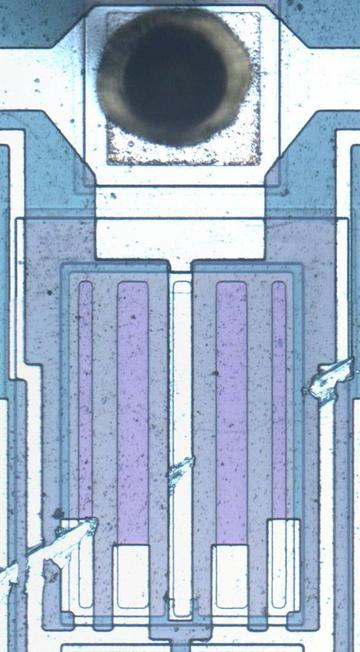Precise small-valued resistors provide a correction factor. 12. The bias voltage circuit generates a stable voltage of one diode drop (about 800 mV) from Q4's collector; this voltage biases the op amps. The tricky part is how to keep the power supply voltage from influencing this voltage or the Zener voltage.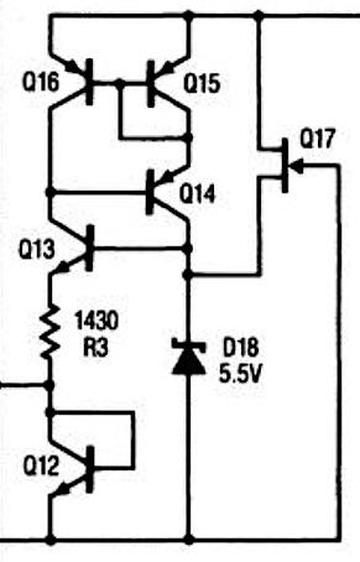The bias generation circuit, from the datasheet. The idea is that the Zener diode puts 5.5 volts on the base of Q13. The voltage across R3 will be two diode drops lower (2.8 V) due to Q13 and Q12. This yields a fixed current of 2.8 V / 1430 Ω = 2 mA through Q4, resulting in a stable voltage drop across Q12 and a stable output. But a Zener's voltage fluctuates a bit with current, so the clever part is how the Zener's current is kept stable. Transistors Q14, Q15, and Q16 form a current mirror, so the current through the Zener will match the current through the resistor, which is 2 mA. Thus, the Zener voltage keeps the resistor current and output voltage stable, while the resistor current keeps the Zener stable. The final piece of the puzzle is the FET Q17, which provides a tiny current through the Zener to start the feedback cycle. HP Nanoprocessor part II: Reverse-engineering the circuits from the masks In 1974, Hewlett-Packard developed a microprocessor for control applications in their products, from floppy disk drives to voltmeters. This simple processor was a step down from the typical microprocessor—it didn't even support addition or subtraction1—so it was called the Nanoprocessor. The Nanoprocessor's key features were its low cost and high speed: compared against the contemporary Motorola 6800, the Nanoprocessor cost$15 instead of $360 and performed control tasks an order of magnitude faster. This processor remained obscure for decades until its designer, Larry Bower, recently donated the chip's masks and documentation to The CPU Shack, who scanned the masks and wrote about the Nanoprocessor. After Antoine Bercovici stitched together the images,2 I wrote a Nanoprocessor overview article based on them. This blog post is part two, where I discuss some of the Nanoprocessor circuitry in detail, reverse-engineering it from the masks. These functional blocks are interesting to study because the Nanoprocessor strips its implementation down to the minimum, while still remaining a useful microprocessor.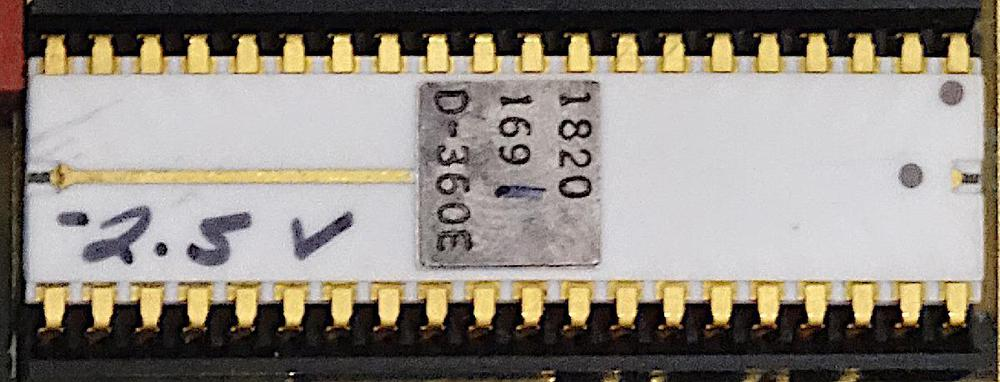The HP Nanoprocessor, part number 1820-1691. Note the hand-written bias voltage "-2.5 V", which varies from chip to chip. The last digit (1) of the part number is also hand-written, indicating the speed of the chip. Photo courtesy of Marc Verdiell. Inside the Nanoprocessor Like most processors of that era, the Nanoprocessor was an 8-bit processor. However, it didn't support RAM,3 but ran code from an external 2-kilobyte ROM. It contained 16 8-bit registers, more than most processors and enough to make up for the lack of RAM in many applications. The Nanoprocessor had 48 instructions, a considerably smaller instruction set than the Motorola 6800's 72 instructions. However, the Nanoprocessor included convenient bit set, clear, and test operations, which other processors of that era lacked. It also had multiple I/O instructions supporting both I/O ports and general-purpose I/O pins, making it easy to control devices with the Nanoprocessor.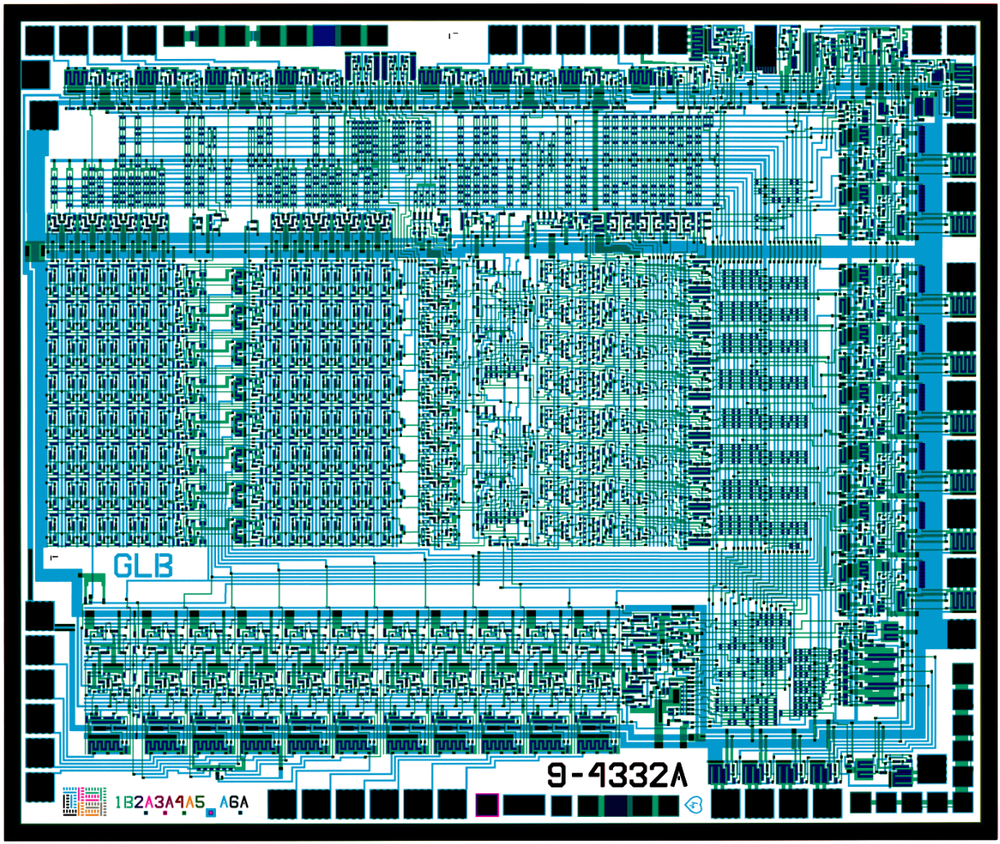Combined masks from the Nanoprocessor. Click for larger image. Files courtesy of Antoine Bercovici using scans from The CPU Shack. The mask image above shows the simplicity of the Nanoprocessor. The blue lines show the metal wiring on top of the chip, while the green shows the doped silicon underneath. The black squares around the outside are the 40 pads for connection to the IC's external pins. The small black regions inside the chip are transistors; if you squint, you should be able to count 4639 of them.4 The block diagram below shows the internal structure of the Nanoprocessor. The 16 storage registers are in the middle. The comparator allows two values to be compared for conditional branches. The Control Logic Unit performs increments, decrements, shifts, and bit operations on the accumulator, lacking the arithmetic and logical operations of a standard Arithmetic/Logic Unit (ALU). The program counter (right) fetches an instruction into the instruction register (left); interrupts and subroutine calls each have a one-entry stack for the return address.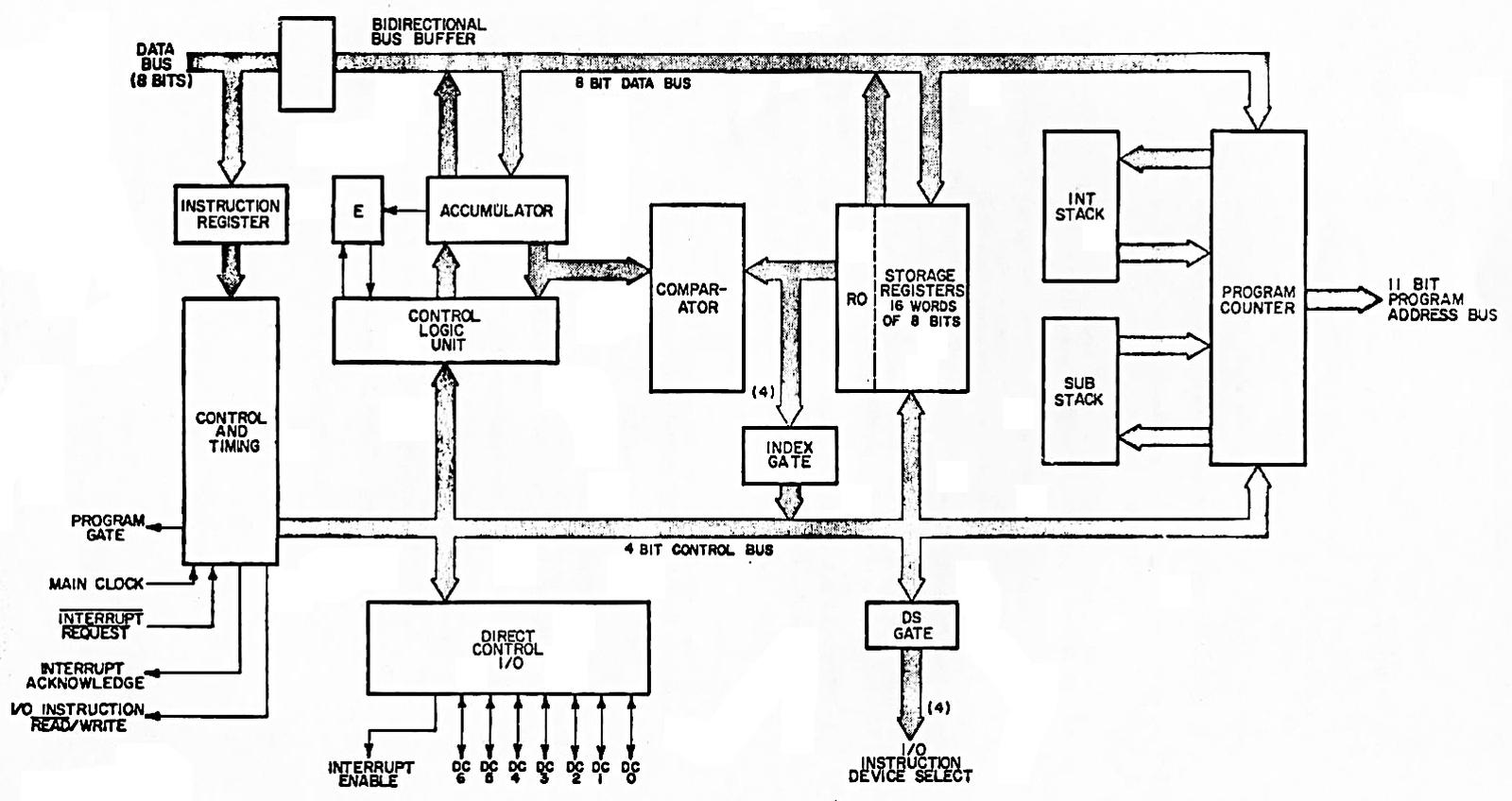Block diagram, from the Nanoprocessor User's Guide. I should emphasize that despite its simplicity5 and lack of arithmetic, the Nanoprocessor is not a "toy" processor that just toggles some control lines, but a fast and capable processor used for complex tasks. The HP 98035 real-time clock module, for instance, uses the Nanoprocessor to parse two dozen different ASCII command strings, as well as activities such as calculating the number of days in each month. Registers The die photo below shows that much of the Nanoprocessor's die is occupied by its 16 registers. These registers communicate with the rest of the chip via the data bus. Circuitry above the registers selects a particular register. Register R0, on the right, is next to the comparator, which will be important later.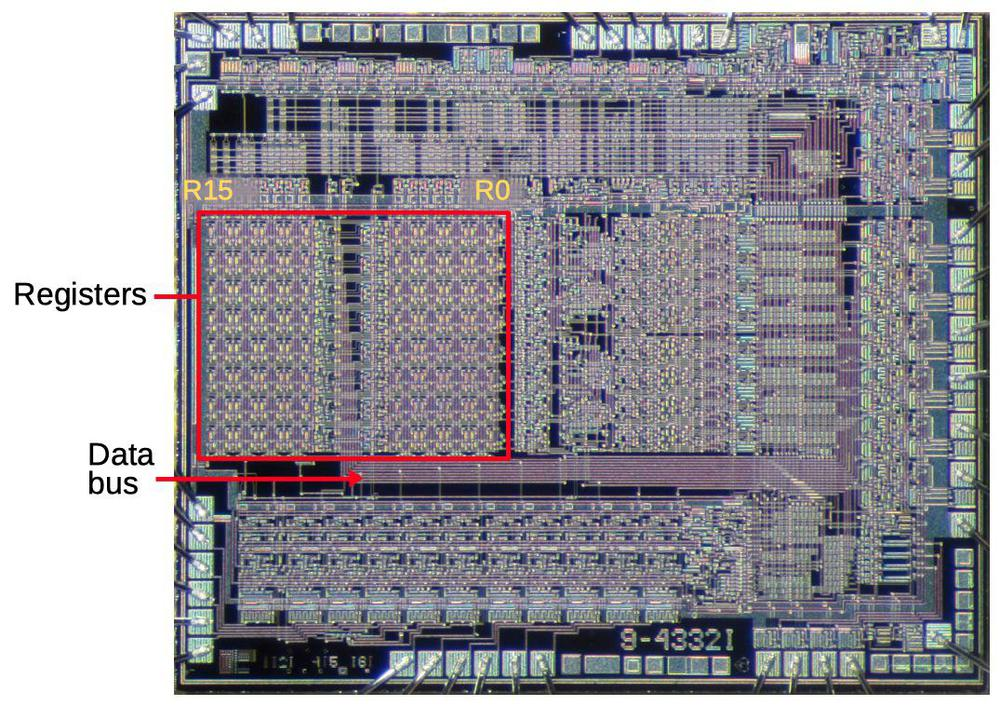The registers take up a large fraction of the Nanoprocessor's die. Underlying die photo by Pauli Rautakorpi, CC BY 3.0. The building block for the registers is two inverters in a feedback loop, storing a single bit as shown below. If the top wire has a 0, the right inverter will output a 1 to the bottom wire. The left inverter will then output a 0 to the top wire, completing the cycle. Thus, the circuit is stable and will "remember" the 0. Likewise, if the top wire is a 1, this will get inverted to a 0 at the bottom wire, and back to a 1 at the top. Thus, this circuit can store either a 0 or a 1, forming a 1-bit memory.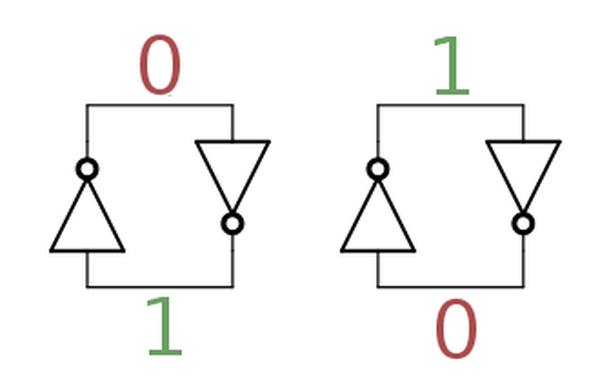Two inverters implement a stable loop that stores a bit. The diagram below shows how this two-inverter storage is implemented on the die. The left shows the physical layout, from the mask images. The layout is optimized to make the cell as small as possible. Blue lines indicate the metal layer, while green is the silicon layer. The schematic in the middle shows the corresponding transistor circuitry. Each inverter is formed from a pair of transistors, as shown on the right. The top and bottom transistors are "pass transistors", providing access to the storage cell.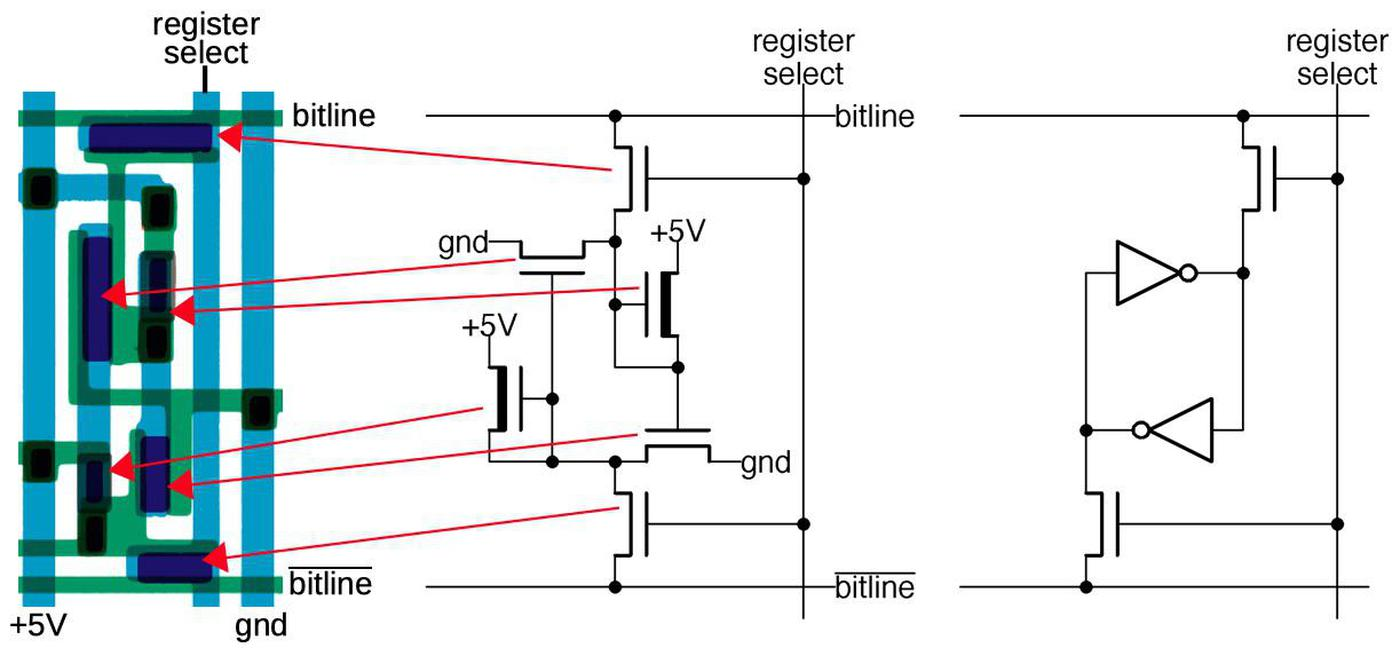One bit of storage in the Nanoprocessor. Each bit is implemented by 6 transistors (also known as a 6T SRAM cell). The register set is built from a matrix of these bit cells. The register select line selects one register (one column) for reading or writing. When selected, the top and bottom pass transistors connect the inverters to the corresponding horizontal bitlines. For a read operation, the top bitline provides the value stored in the cell; there are eight pairs of bitlines for the eight bits in a register. For a write operation, the value is applied to the upper bitline and the inverted value is applied to the lower bitline. These values overpower the signals from the inverters, forcing the inverters to the desired value and storing the bit. Thus, the grid of horizontal bitlines and vertical select lines allows a particular register to be read or written. Instruction decoding The instruction decoding circuitry is responsible for taking a binary instruction code (such as 01101010) and determining what instruction it is ("Load accumulator from register 10" in this case). Compared to many processors, the Nanoprocessor's instructions are pretty simple: it has relatively few instructions (48) and the opcode is always one byte long. The diagram below shows that instruction decoding logic (red) takes up a large fraction of the chip. The instruction register (green), is a set of eight latches holding the current instruction. The instruction register is next to the data pins, which provide the instruction from the ROM. This section will focus on the decoding circuit in the yellow box.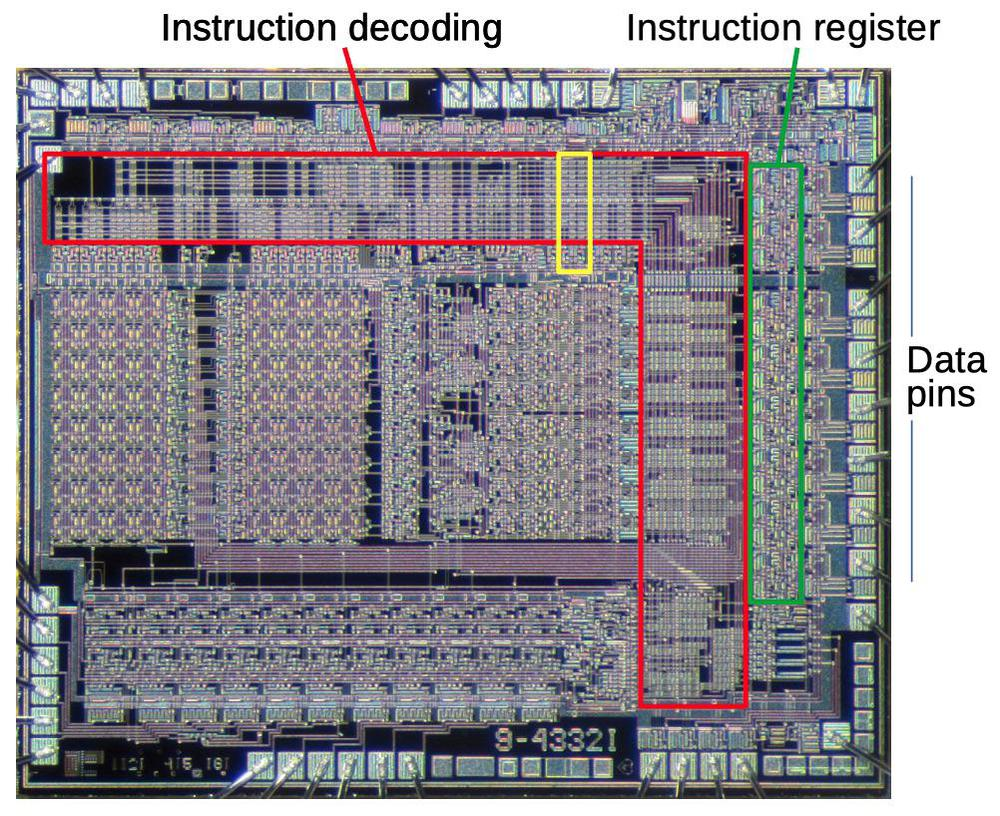A large part of the chip is devoted to instruction decoding (red). This section will focus on the circuit highlighted in yellow. Underlying die photo by Pauli Rautakorpi, CC BY 3.0. Decoding is done by NOR gates; each NOR gate detects a particular instruction or group of instructions. The NOR gates take instruction bits or their complements as inputs. When all inputs are zero, the NOR gate indicates a match. This allows matching against the entire instruction or part of the instruction. For instance, the "Load accumulator from register R" instruction has the binary format "0110rrrr", where the last four bits indicate the desired register. A NOR gate (bit7 + bit6' + bit5' + bit4)' will match instructions of that form. The nice thing about structuring the instruction decoder in this way is that it can be built from compact, regular circuits, often called a PLA.6 The idea is to make a matrix with input signals running horizontally and outputs vertically. Each intersection can have a transistor, making the input signal part of the gate; or no transistor, ignoring that input signal. The result is tightly-packed NOR gates. The diagram on the right below zooms in on the three decoders highlighted in yellow above. The schematic corresponds to the leftmost decoder; note the correspondence between transistors in the schematic and the pink transistor blobs in the layout. The idea is that if any input energizes a transistor, the transistor will pull the output to ground. Otherwise, the output is pulled high by the resistor. The inverters at the bottom amplify the signal, providing enough current to drive all eight slices of the accumulator.7 Curiously, the layout uses pairs of transistors, both connected between ground and the output; I don't see the advantage over the straightforward approach of using a single transistor. In any case, note how the PLA-style matrix provides a dense layout for the decoders.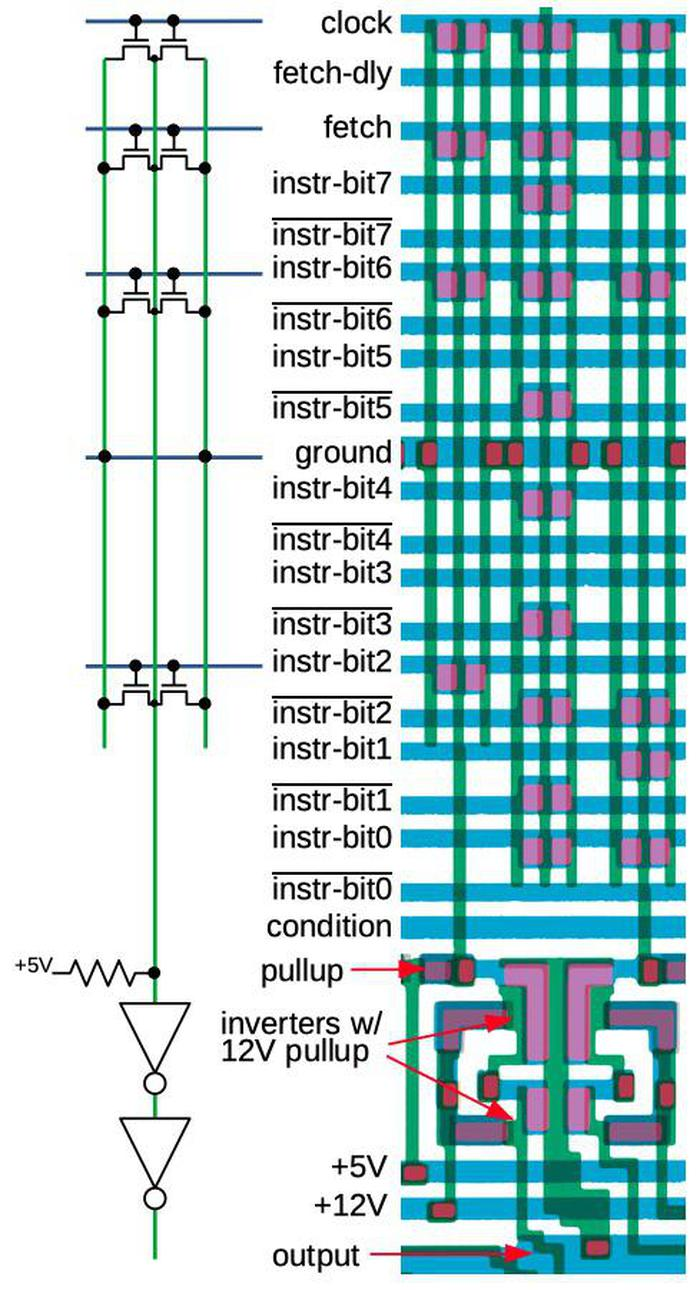This diagram shows one of the decoder circuits in the Nanoprocessor. The schematic corresponds to the leftmost decoder of the three shown on the right. This particular circuit generates the increment/decrement signal that is fed into the accumulator circuit. This circuit matches when the clock, fetch, instruction bit 6, and instruction bit 2 are all low, so it matches instructions of the form x0xxx0xx during execute phase. These instructions include "Increment Binary" (00000000), "Increment BCD" (00000010), "Decrement Binary" (00000001) and "Decrement BCD" (00000011).8 Comparator An important circuit in the Nanoprocessor is the comparator that determines if the accumulator A is greater, less than, or equal to register R0. The comparator uses a simple but clever circuit to compare these two values. The algorithm is essentially to compare the two numbers starting with the most significant bits. As long as the bits are equal, keep moving to the less significant bits. The first difference between the two numbers determines which one is greater. (For instance, with 10101010 and 10100111, the highlighted bits determine that the first number is greater.) This algorithm is implemented with eight stages, one for each bit, starting with the most significant bits at the bottom. Each stage (below) consists of two symmetrical parts: one determines if A > R0, while the complementary one determines if A < R0. If the numbers are equal so far, but the two bits are different at this stage, the stage generates the greater than or less than signal. Otherwise, it passes along the decision of the lower stage. The topmost stage outputs the final decision. Note that the comparator provides an equality test "for free"; if the output isn't greater than or less than, the two numbers are equal.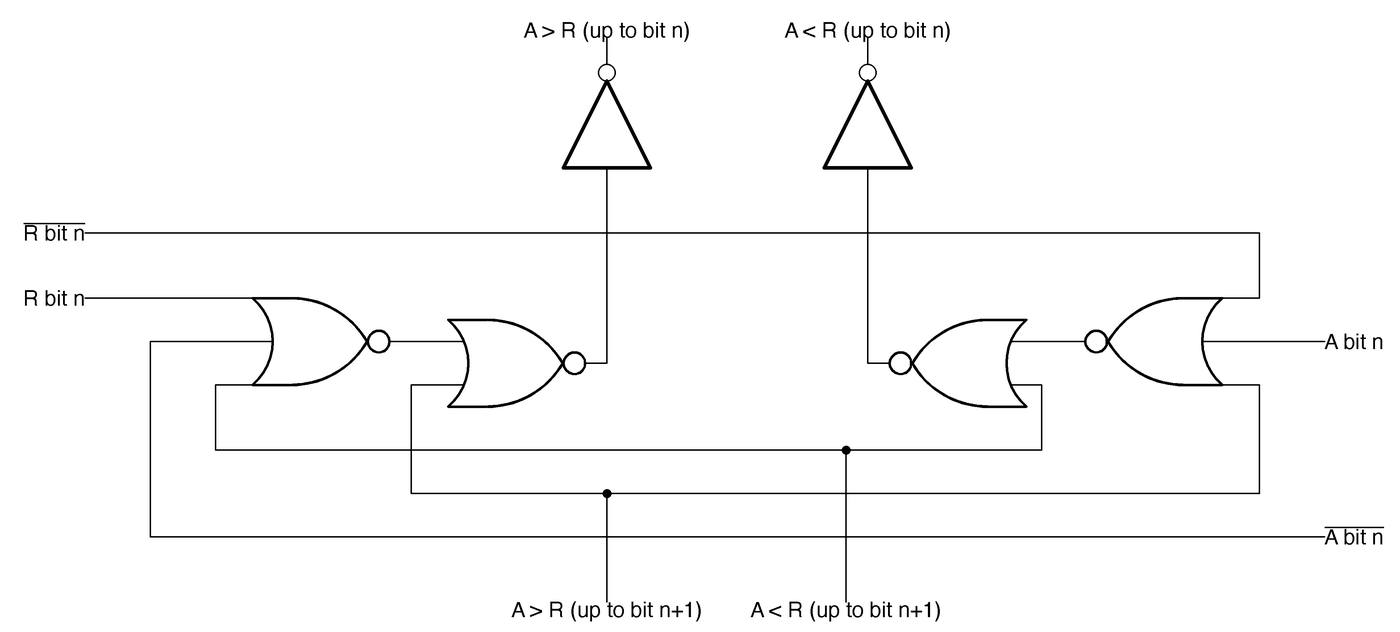One stage of the 8-bit comparator. The diagram below shows the physical layout of two comparator stages. One clever feature of the comparator's layout is that it sits between register 0 on the left and the accumulator on the right, minimizing wiring. The comparator accesses register 0 directly, without going through the regular path of the register selection and the data bus.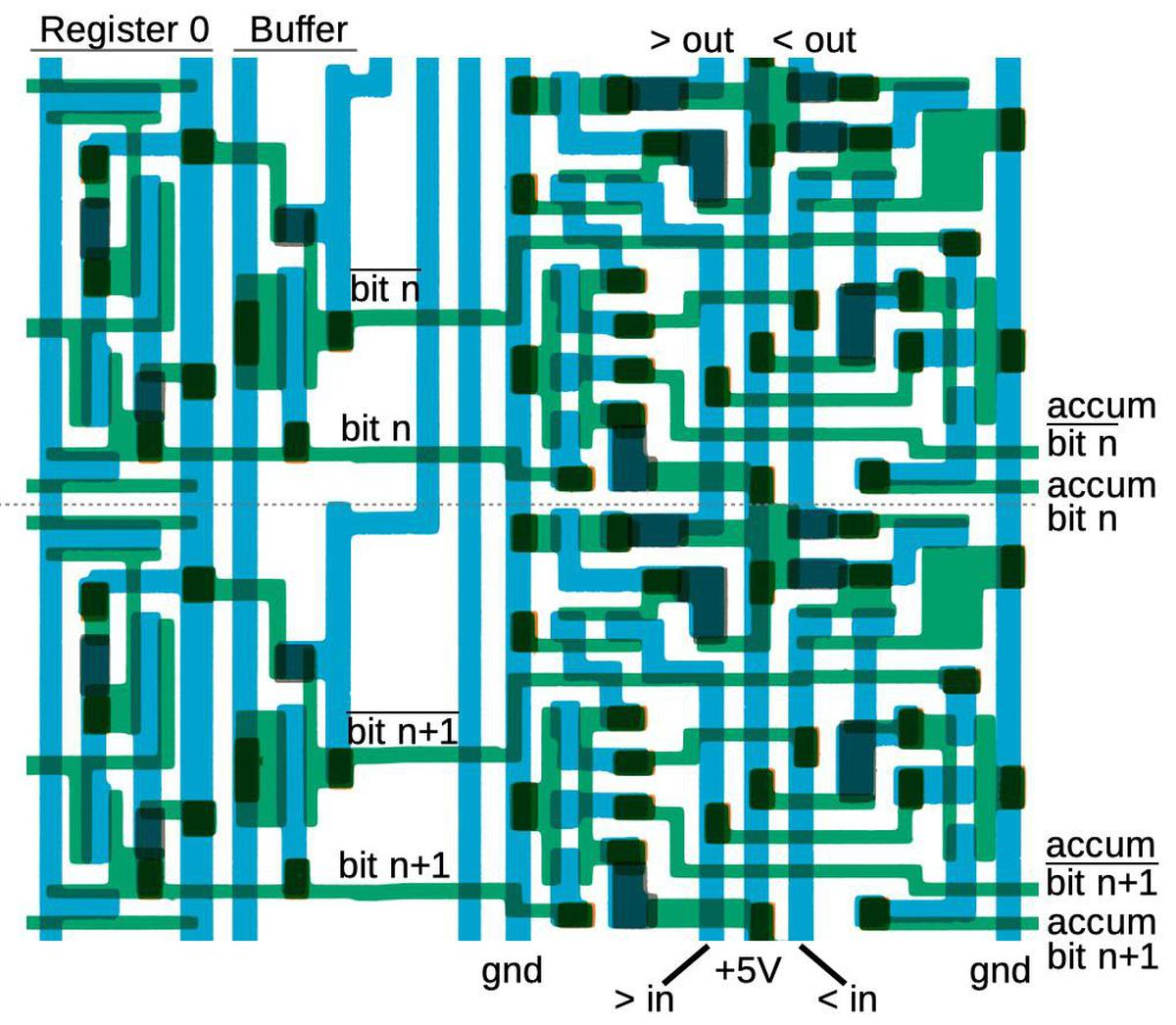Two stages of the comparator, as it appears in the masks. The Nanoprocessor's conditional branch instructions can test the comparator outputs.9 The branch circuitry is fairly straightforward: several bits of the branch instruction select the particular test via a multiplexer. Then bit 7 of the instruction selects "branch if true" versus "branch if false". Unlike most processors, the Nanoprocessor doesn't provide branches to an arbitrary address. Instead, it skips two instruction bytes if the condition is satisfied. (Typically these two bytes would hold a jump to the desired target, but sometimes hold other instructions.) The skip circuit is simple: the program counter incrementer (described below) is triggered a second time, but increments by two instead of one, skipping two instructions. Thus, the Nanoprocessor implements an extensive set of conditional tests with a relatively small amount of circuitry. Accumulator and Control Logic Unit The accumulator is the special 8-bit register that stores the byte currently being processed. Operations on the accumulator are performed by the Control Logic Unit (CLU), which the manual calls "the heart of the Nanoprocessor". The CLU is the equivalent of the Arithmetic/Logic Unit (ALU) in most processors, except it doesn't perform arithmetic or logic operations. The CLU is not quite as useless as it sounds, though. It can increment or decrement the accumulator, both in binary and binary coded decimal (BCD). (Binary coded decimal stores two decimal digits per byte. This is very useful for decimal I/O or displays.) The CLU can also complement or clear the accumulator, or set or clear a specific bit. Finally, it supports left and right shift operations.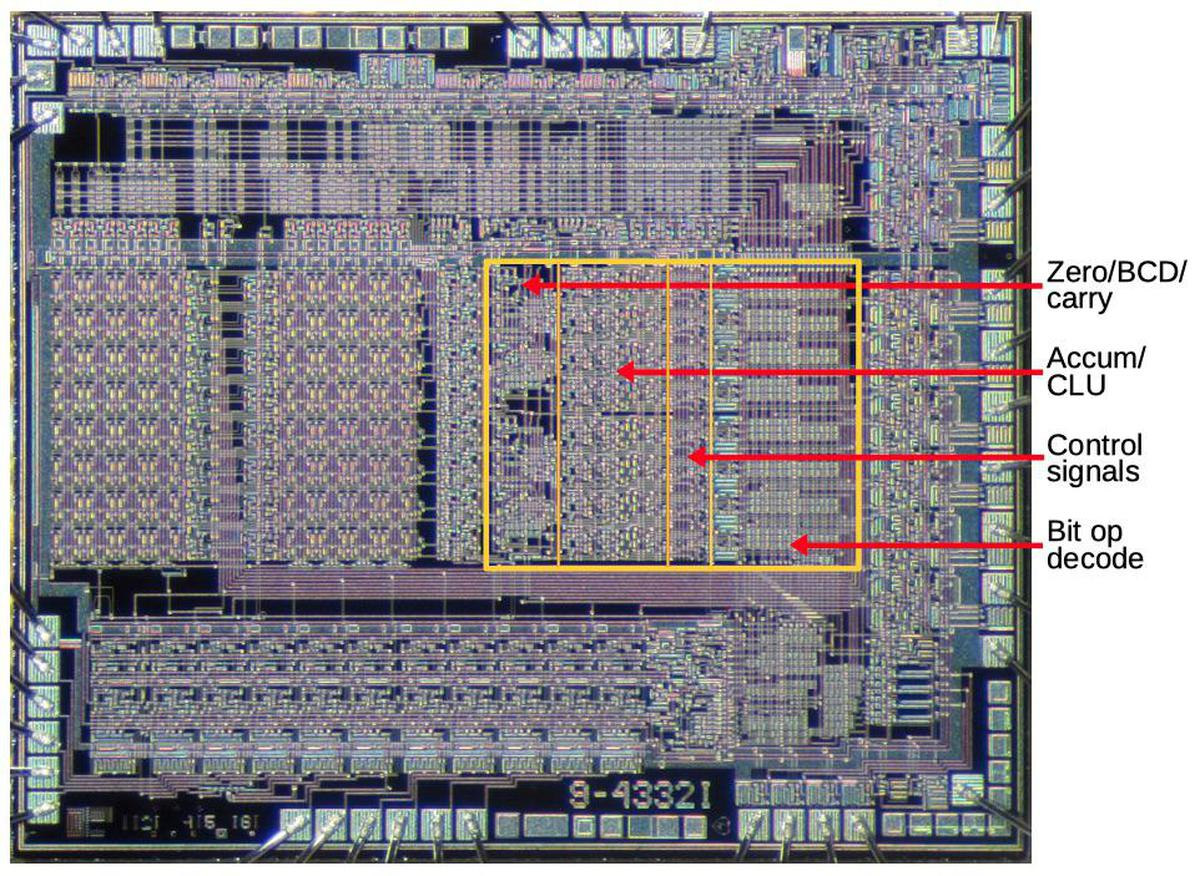Circuitry related to the accumulator. Underlying die photo by Pauli Rautakorpi, CC BY 3.0. The diagram above shows the layout of the accumulator and CLU. The first region has miscellaneous circuitry to detect a zero value; support BCD by detecting a 9 digit, for instance; and provide carry-skip, fast carry generation from the lower 4 bits. I won't discuss this in more detail, but note the irregular layout of this circuitry. The second region holds the main accumulator and CLU circuitry; I will discuss this in detail below. The third region distributes control signals from the decode logic above to the eight accumulator slices. Finally, the last region holds instruction decoding logic to decode bit operations and signal the appropriate accumulator slice. The main part of the accumulator/CLU consists of 8 slices, one for each bit, with the lowest bit at the top. I will discuss four circuits in each slice: the incrementer/decrementer's carry generation, the incrementer/decrementer's bit generation, the multiplexer to select the new accumulator value, and the latch that holds the accumulator's value. Each slice of the incrementer/decrementer (below) is implemented by a half adder. The direction of the incrementer/decrementer circuit depends on the opcode: a 0 in the opcode's low bit indicates an increment, while a 1 in the opcode's low bit indicates a decrement. The carry circuit on the left below generates the carry-out signal. For an increment, there is a carry-out if there is a carry-in and the current bit is 1 (since it will be incremented to binary 10). For decrement, the carry line indicates a borrow, rather than a carry, so there is a carry-out if there is a carry-in (i.e. a borrow) and the current bit is 0, triggering a borrow.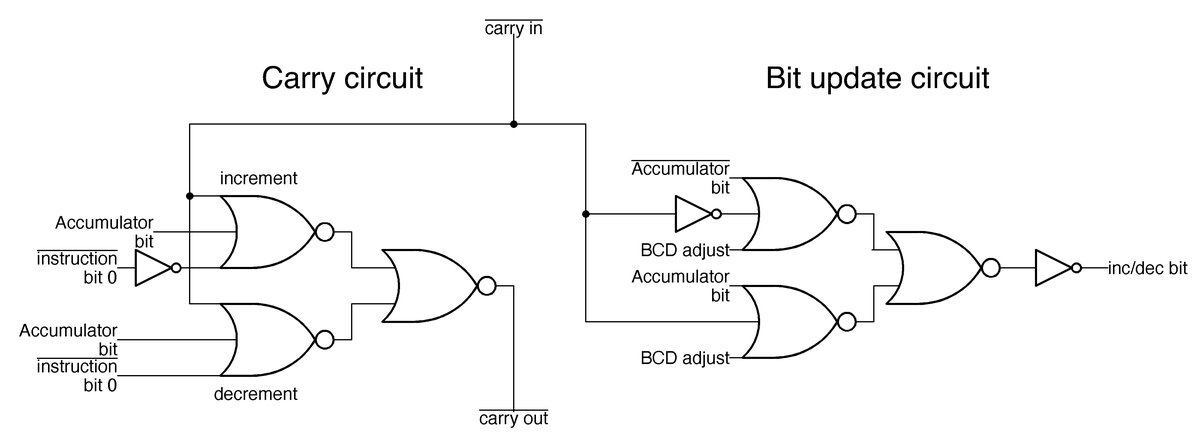One slice of the incrementer/decrementer circuit. The circuit on the right above updates the current bit when incrementing or decrementing. The current bit is flipped if there is a carry-in, essentially an XOR implemented by three NOR gates. One complication is the adjustment for BCD (binary-coded decimal). For a BCD increment operation, a carry occurs when incrementing a 9 digit, while for a BCD decrement, a 0 digit is decremented to 9, not to binary 1111. The different accumulator operations are provided by the multiplexer below. Depending on the operation, one pass transistor will be activated, selecting the desired value. For instance, for an increment/decrement operation, the top transistor selects the output from the increment/decrement circuit described above. This transistor is activated by the instruction decoder described earlier that matches an increment/decrement instruction. Similarly, a shift-right instruction activates the shift-right pass transistor, feeding accumulator bit n+1 into each accumulator slice to shift the value.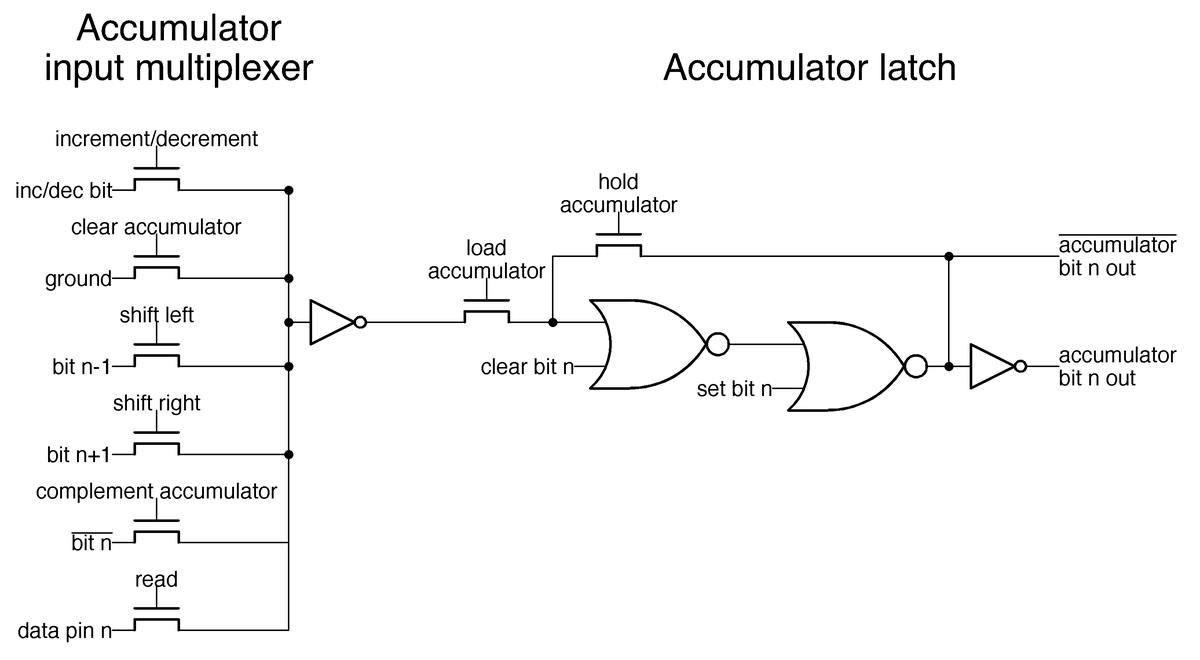Schematic of the latch holding one bit of the accumulator, along with the multiplexer that selects an input to the accumulator. The latch above stores one bit of the accumulator. When the hold accumulator transistor is activated, the two NOR gates form a loop, holding the value. But when the load accumulator transistor is activated instead, the accumulator loads its value from the multiplexer. The clear bit n and set bit n lines allow instructions to modify individual bits of the accumulator; the multiplexer, in comparison, updates all accumulator bits at once. Program counter and addressing Another large block of circuitry is the 11-bit program counter in the lower left of the Nanoprocessor, which I'll describe briefly. This block also includes a latch to hold the return address for a subroutine call and a second latch to hold the program counter after an interrupt. (You can think of these as one-entry stacks.) The program counter includes an incrementer to advance it to the next instruction. This incrementer can also increment by two, allowing conditional branch instructions to skip over two instructions. (Increment-by-two is implemented by incrementing bit 1 instead of bit 0.) To improve the performance of the incrementer, it has a carry-skip feature; if the bottom six bits are all 1, it will increment bit 6 immediately without waiting for the carry to propagate through the low-order bits. Control and timing The final piece of the Nanoprocessor is the control circuitry. Compared to other microprocessors, the Nanoprocessor's control circuitry is almost trivial: the processor alternates between fetch and execute cycles (with the occasional interrupt). The control circuitry is not much more than a couple of flip flops and gates, so I won't say more about it. Conclusions The diagram below summarizes the main functional blocks of the Nanoprocessor. The Nanoprocessor achieves a dense layout for these blocks, much better than I would expect from its obsolete metal-gate technology.10 Reverse-engineering shows that these functional blocks are implemented with simple but carefully-designed circuits.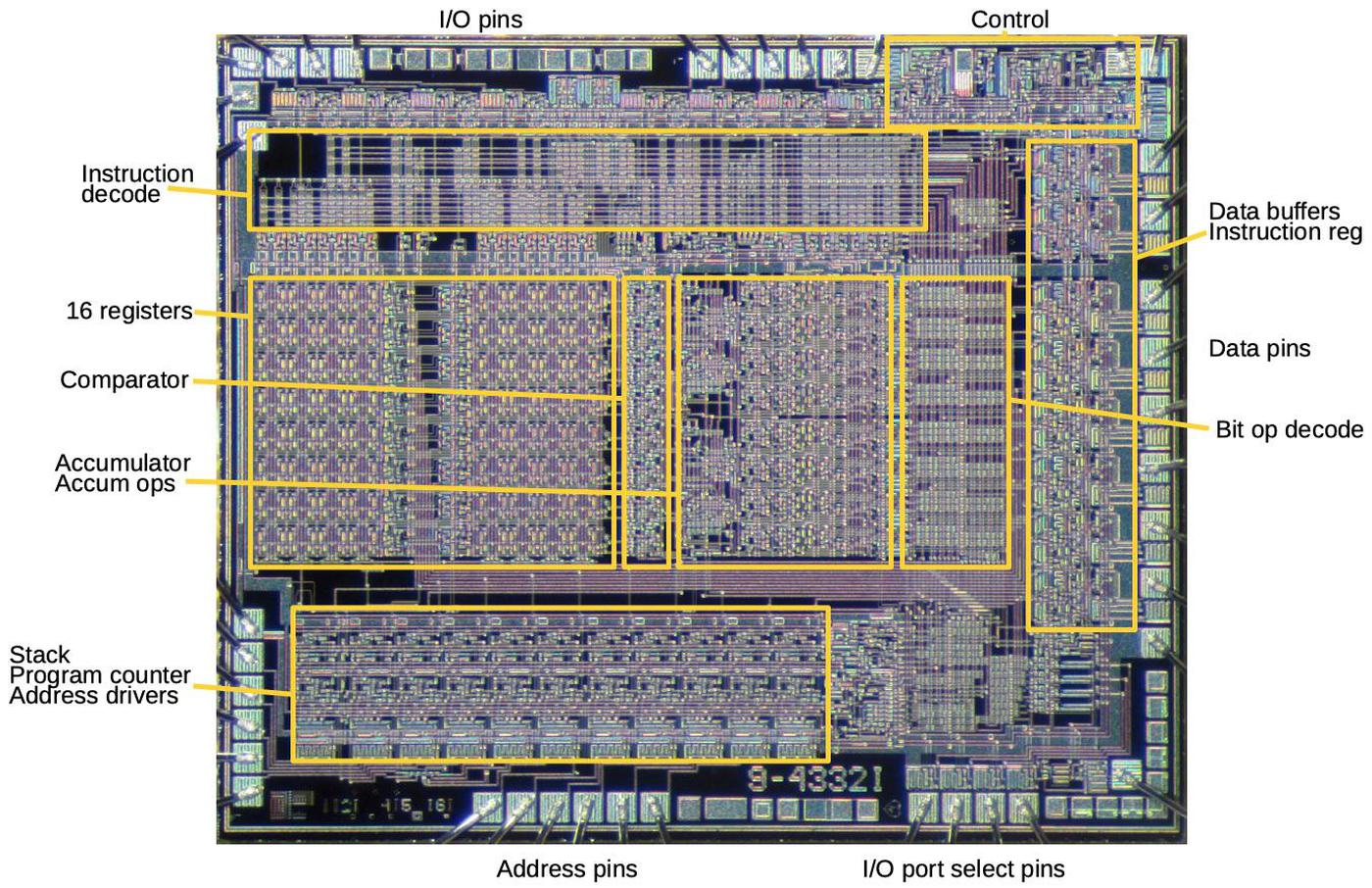Functional components of the HP Nanoprocessor, based on my reverse-engineering. Underlying die photo by Pauli Rautakorpi, CC BY 3.0. The Nanoprocessor is an unusual processor. My first impression was that it wasn't even a "real processor," since it lacked basic arithmetic functionality. However, after studying it, I'm more impressed. Its simple design allows it to operate faster than other processors of the time. The instruction set is more capable than it appears at first. Hewlett-Packard used the Nanoprocessor in many products in the 1970s and 1980s, in roles that were more complex than you'd expect, such as parsing strings and performing calculations. Now, with the masks released by The CPU Shack, we can learn the secrets of the circuits that made the Nanoprocessor work.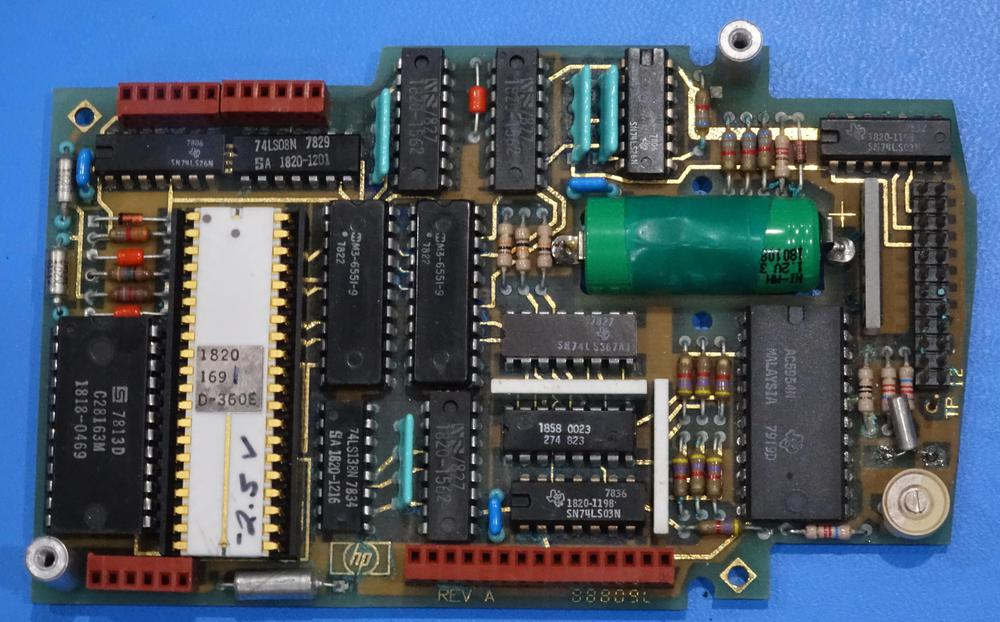Nanoprocessor (white chip) as part of an HP clock module. Note the hand-written voltage on the chip; each chip required a different bias voltage. Photo courtesy of Marc Verdiell. Follow me on Twitter at @kenshirriff for updates on my blog posts. I also have an RSS feed. Thanks to Antoine Bercovici for scanning and remastering the masks, Larry Bower for the donation, and John Culver at The CPU Shack for sharing the donation. Notes and references 1. Although it lacks an addition instruction, the Nanoprocessor can add numbers (slowly) through repeated increment and decrement operations (which it supports). (The code for the HP real-time clock module does this.) Other applications, such as the HP voltmeter, added external ALU chips (74LS181) to perform fast addition; these were accessed as I/O devices. (With Turing-completeness, of course, the Nanoprocessor can theoretically do anything from floating-point functions to Crysis; it will just be very slow.) 2. The mask images can be downloaded here (warning: 122 MB PSD file). 3. The Nanoprocessor doesn't have instructions to support RAM, since it is designed for control applications that typically don't need much storage. However, some Nanoprocessor applications use RAM, treating RAM as an I/O device. The address is written to one I/O port and the data byte is read or written from another port. 4. By my count, the Nanoprocessor has 4639 transistors. The instruction decoder is constructed from pairs of small transistors for layout reasons; combining these pairs yields 3829 unique transistors. Of these, 1061 act as pull-ups, while 2668 are active. In comparison, the 6502 has 4237 transistors, of which 3218 are active. The 8008 has 3500 transistors and the Motorola 6800 has 4100 transistors. 5. Making an FPGA version of the Nanoprocessor would probably be a fun project since the Nanoprocessor is about as simple as you can make a real, commercial processor. The User's Guide explains the instructions and has sample code that could be executed. 6. Building the decoder out of an array of NOR gates decoding was common in early microprocessors, for instance the 6502, because it could be constructed in a regular, compact form. It's often called a PLA (Programmable Logic Array), even though a PLA is supposed to have two layers of logic. 7. Note that the inverters in the instruction decoder are pulled up to 12 volts, rather than 5 volts. The reason is that the Nanoprocessor uses metal-gate transistors, rather than the more advanced silicon-gate transistors of other microprocessors of the era. Metal-gate transistors have the disadvantage of a higher threshold voltage, which means the output of a transistor is considerably lower than the gate voltage. The output from a regular inverter is too low to drive the gate of a pass transistor, since the output will be another threshold voltage below that. The solution is to use the 12 volt supply for the decoder inverters that drive pass transistors in the accumulator. Then, these signals have plenty of voltage to drive pass transistors. In other words, the Nanoprocessor required an extra +12V supply because it used metal-gate transistors instead of the more modern silicon-gate transistors. 8. The illustrated decode circuit matches against instructions x0xxx0xx, so it matches against many more instructions than just the increment and decrement instructions. Why doesn't the circuit match exactly? The reason is that if the accumulator is not being used, it doesn't matter if the increment/decrement signal is activated. By making the match wider, the designers could omit some transistors. The important point is that the circuit rejects other accumulator instructions such as "Clear accumulator" (00000100) or "Load accumulator from register" (0110rrrr). 9. The Nanoprocessor has an extensive set of conditional branches, surprisingly many for a simple processor. You can branch if A > R0, A >= R0, A < R0, A <= R0, A == R0, or A != R0. In additional conditional branches can be done on the accumulator being zero or nonzero, any particular bit of the accumulator being zero or nonzero, the overflow flag being set or not, or a particular general-purpose I/O ("direct control") bit being set or not. 10. The Nanoprocessor used metal-gate transistors, while other microprocessors started using silicon-gate transistors a few years earlier. This may seem like an obscure difference, but it has a huge impact on layout: silicon-gate fabrication added a layer of polysilicon wiring. This makes layout much easier, since you now have two layers for wiring that can cross each other. With just the metal layer for wiring, like the Nanoprocessor, layout is difficult because wires keep getting in the way of each other. In other metal-gate chips that I've examined, the layout is just awful; there's a lot of convoluted wiring to get the signals to each transistor, so the transistor density is low. In comparison, the Nanoprocessor's functional blocks are all carefully designed so the signals all flow together nicely. There's some wasted space, for instance for the data bus, but overall, I'm impressed by the density of the Nanoprocessor's layout. Reverse-engineering the first FPGA chip, the XC2064 A Field-Programmable Gate Array (FPGA) can implement arbitrary digital logic, anything from a microprocessor to a video generator or crypto miner. An FPGA consists of many logic blocks, each typically consisting of a flip flop and a logic function, along with a routing network that connects the logic blocks. What makes an FPGA special is that it is programmable hardware: you can redefine each logic block and the connections between them. The result is you can build a complex digital circuit without physically wiring up individual gates and flip flops or going to the expense of designing a custom integrated circuit.Die photo closeup showing the circuitry for one of the 64 tiles in the XC2064 FPGA. The metal layers have been removed, exposing the silicon and polysilicon transistors underneath. Click for a larger image. From siliconpr0n. The FPGA was invented by Ross Freeman1 who co-founded Xilinx2 in 1984 and introduced the first FPGA, the XC2064. 3 This FPGA is much simpler than modern FPGAs—it contains just 64 logic blocks, compared to thousands or millions in modern FPGAs—but it led to the current multi-billion-dollar FPGA industry. Because of its importance, the XC2064 is in the Chip Hall of Fame. I reverse-engineered Xilinx's XC2064, and in this blog post I explain its internal circuitry (above) and how a "bitstream" programs it.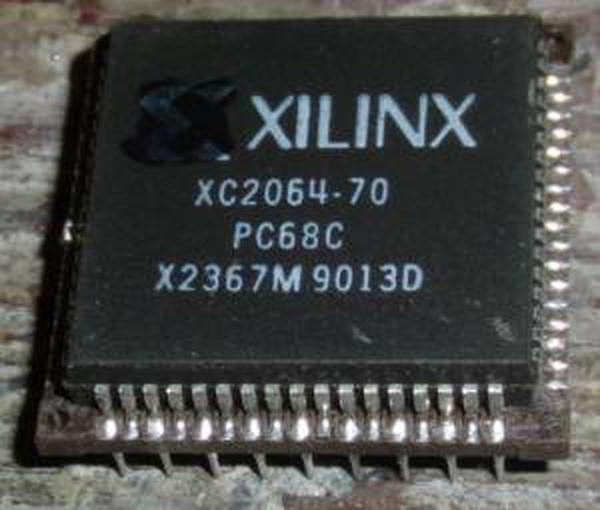The Xilinx XC2064 was the first FPGA chip. Photo from siliconpr0n. Nowadays, an FPGA is programed in a hardware description language such as Verilog or VHDL, but back then Xilinx provided their own development software, an MS-DOS application named XACT with a hefty$12,000 price tag. XACT operated at a lower level than modern tools: the user defined the function of each logic block, as shown in the screenshot below, and the connections between logic blocks. XACT routed the connections and generated a bitstream file that could be loaded into the FPGA.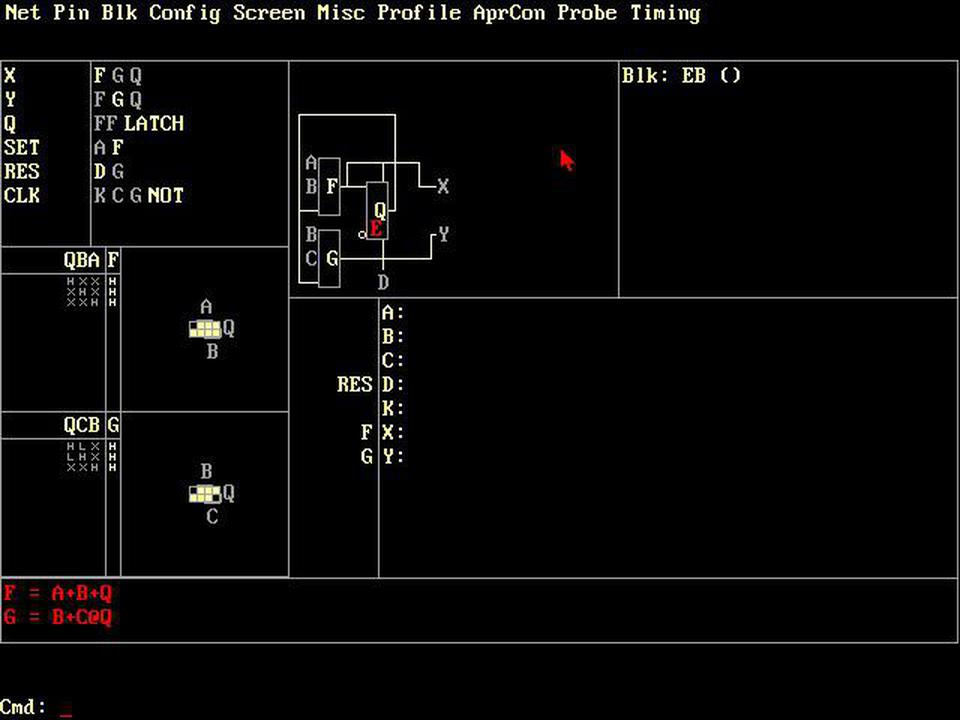Screenshot of XACT. The two lookup tables F and G implement the equations at the bottom of the screen, with Karnaugh map shown above.

An FPGA is configured via the bitstream, a sequence of bits with a proprietary format. If you look at the bitstream for the XC2064 (below), it's a puzzling mixture of patterns that repeat irregularly with sequences scattered through the bitstream. There's no clear connection between the function definitions in XACT and the data in the bitstream. However, studying the physical circuitry of the FPGA reveals the structure of the bitstream data and it can be understood.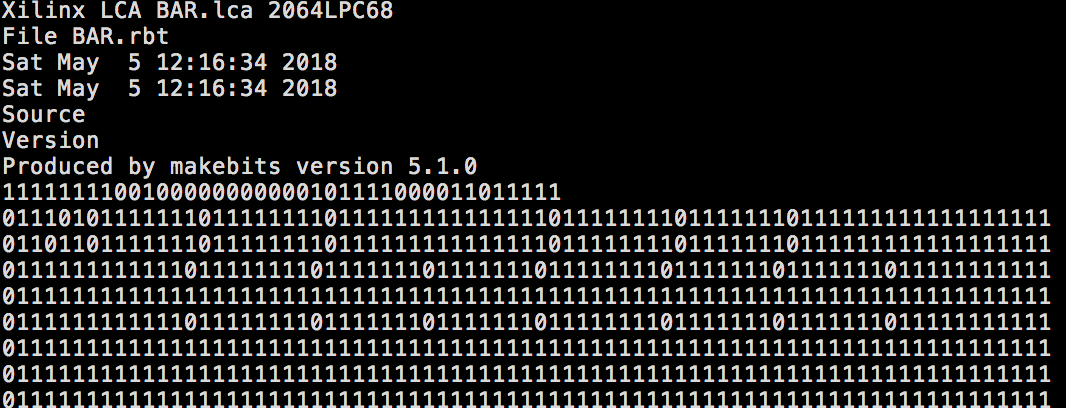Part of the bitstream generated by XACT.

How does an FPGA work?

The diagram below, from the original FPGA patent, shows the basic structure of an FPGA. In this simplified FPGA, there are 9 logic blocks (blue) and 12 I/O pins. An interconnection network connects the components together. By setting switches (diagonal lines) on the interconnect, the logic blocks are connected to each other and to the I/O pins. Each logic element can be programmed with the desired logic function. The result is a highly programmable chip that can implement anything that fits in the available circuitry.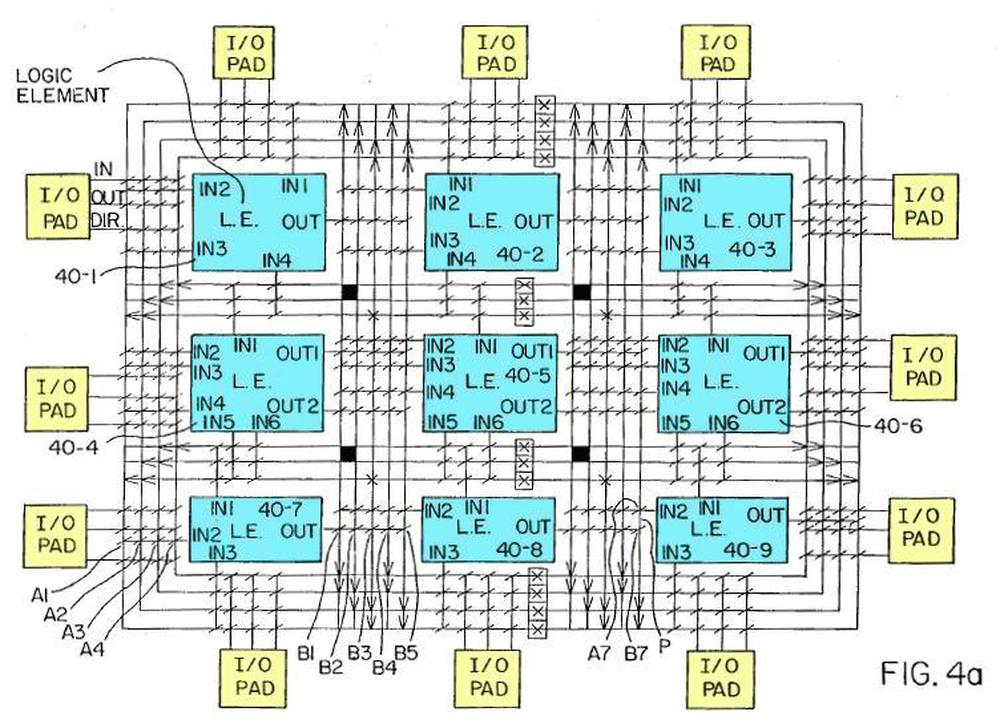The FPGA patent shows logic blocks (LE) linked by an interconnect.

CLB: Configurable Logic Block

While the diagram above shows nine configurable logic blocks (CLBs), the XC2064 has 64 CLBs. The diagram below shows the structure of each CLB. Each CLB has four inputs (A, B, C, D) and two outputs (X and Y). In between is combinatorial logic, which can be programmed with any desired logic function. The CLB also contains a flip flop, allowing the FPGA to implement counters, shift registers, state machines and other stateful circuits. The trapezoids are multiplexers, which can be programmed to pass through any of their inputs. The multiplexers allow the CLB to be configured for a particular task, selecting the desired signals for the flip flop controls and the outputs.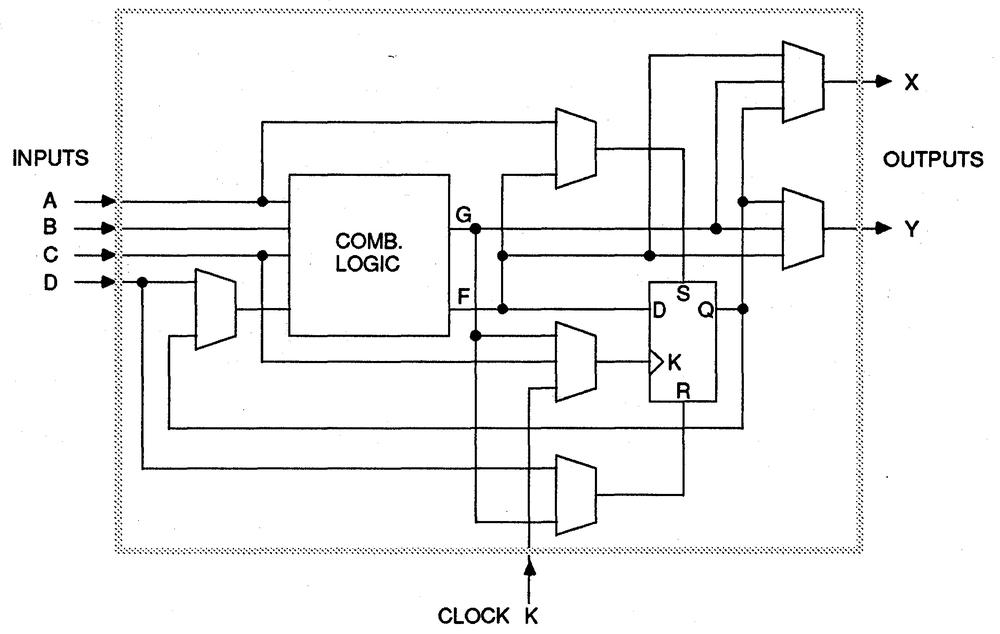A Configurable Logic Block in the XC2064, from the datasheet.

You might wonder how the combinatorial logic implements arbitrary logic functions. Does it select between a collection of AND gates, OR gates, XOR gates, and so forth? No, it uses a clever trick called a lookup table (LUT), in effect holding the truth table for the function. For instance, a function of three variables is defined by the 8 rows in its truth table. The LUT consists of 8 bits of memory, along with a multiplexer circuit to select the right value. By storing values in these 8 bits of memory, any 3-input logic function can be implemented.4

The interconnect

The second key piece of the FPGA is the interconnect, which can be programmed to connect the CLBs in different ways. The interconnect is fairly complicated, but a rough description is that there are several horizontal and vertical line segments between each CLB. CLB. Interconnect points allow connections to be made between a horizontal line and a vertical line, allowing arbitrary paths to be created. More complex connections are done via "switch matrices". Each switch matrix has 8 pins, which can be wired together in (almost) arbitrary ways.

The diagram below shows the interconnect structure of the XC2064, providing connections to the logic blocks (cyan) and the I/O pins (yellow). The inset shows a closeup of the routing features. The green boxes are the 8-pin switch matrices, while the small squares are the programmable interconnection points.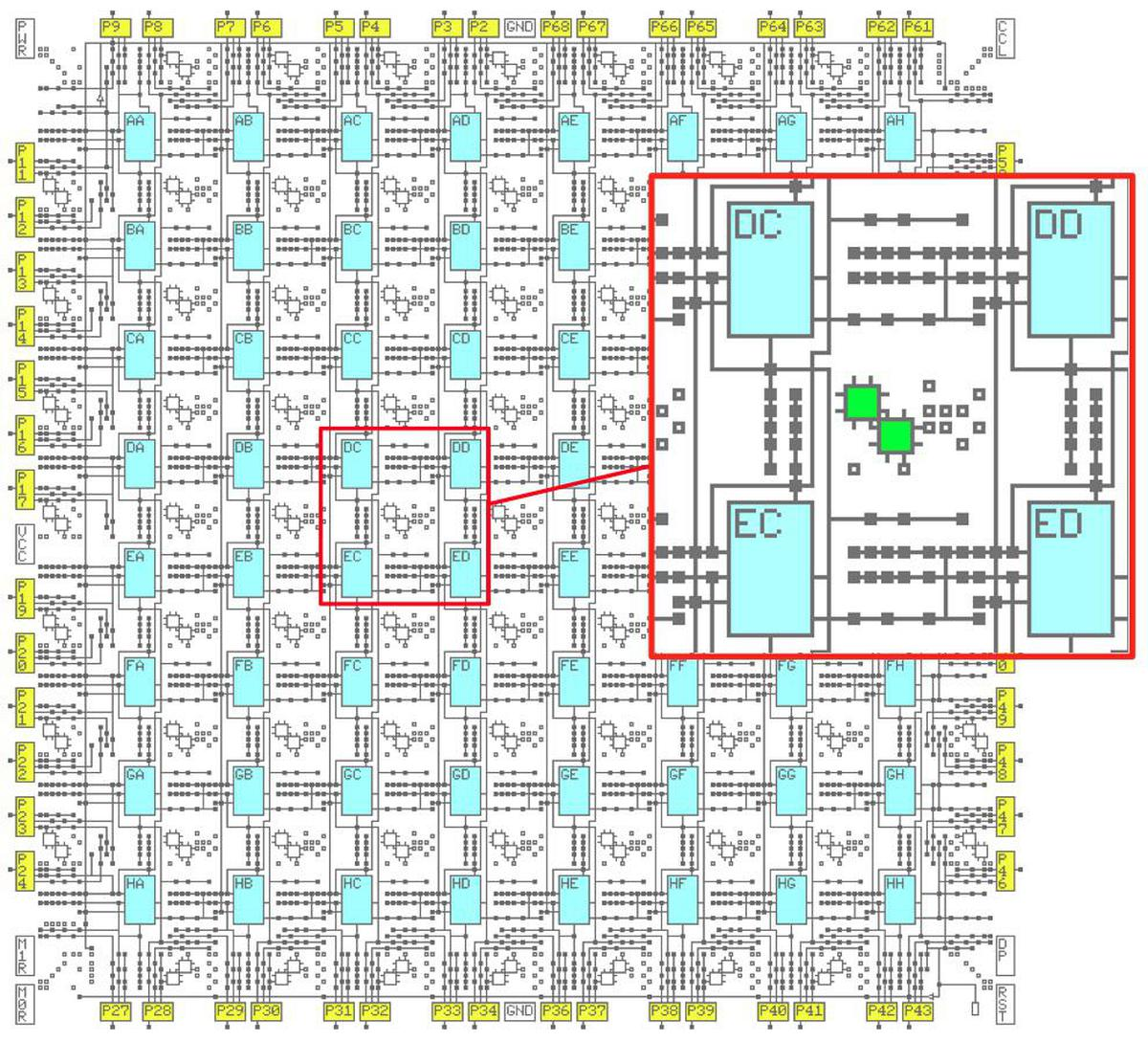The XC2064 FPGA has an 8 by 8 grid of CLBs. Each CLB has an alphabetic name from AA to HH.

The interconnect can wire, for example, an output of block DC to an input of block DE, as shown below. The red line indicates the routing path and the small red squares indicate activated routing points. After leaving block DC, the signal is directed by the first routing point to an 8-pin switch (green) which directs it to two more routing points and another 8-pin switch. (The unused vertical and horizontal paths are not shown.) Note that routing is fairly complex; even this short path used four routing points and two switches.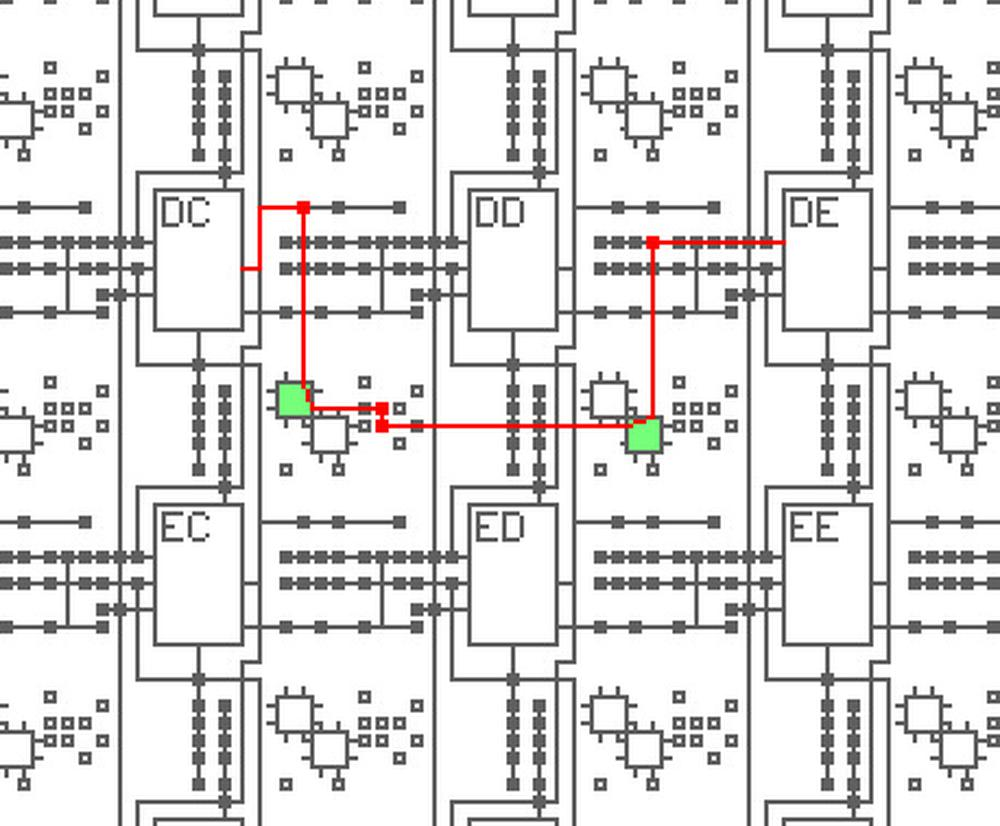Example of a signal routed from an output of block DC to block DE.

The screenshot below shows what routing looks like in the XACT program. The yellow lines indicate routing between the logic blocks. As more signals are added, the challenge is to route efficiently without the paths colliding. The XACT software package performs automatic routing, but routes can also be edited manually.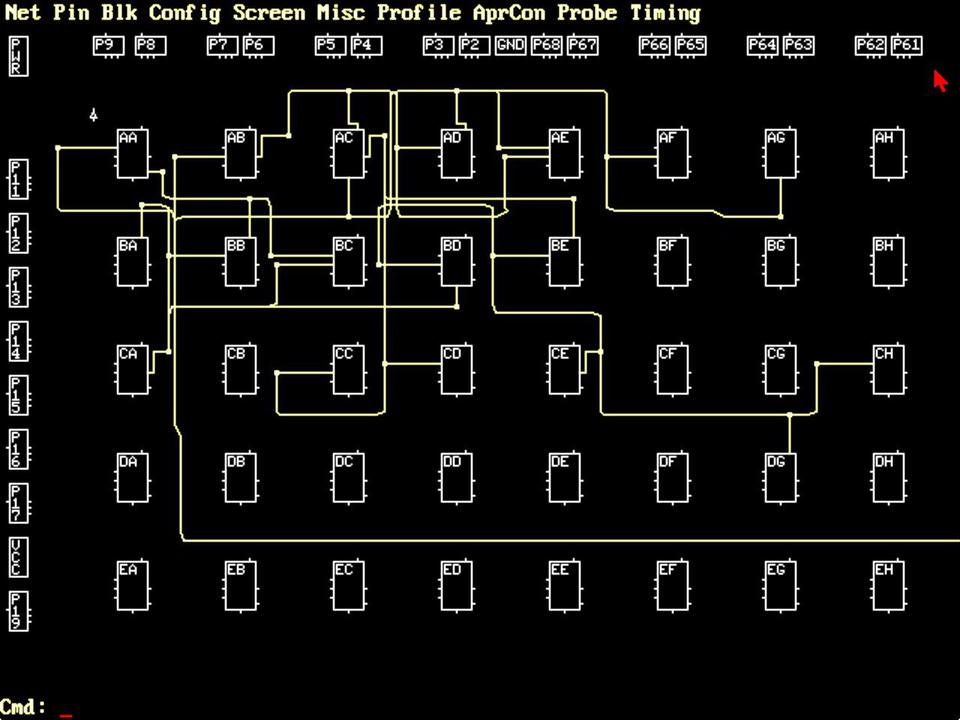Screenshot of the XACT program. This MS-DOS program was controlled via the keyboard and mouse.

The implementation

The remainder of this post discusses the internal circuitry of the XC2064, reverse-engineered from die photos.5 Be warned that this assumes some familiarity with the XC2064.

The die photo below shows the layout of the XC2064 chip. The main part of the FPGA is the 8×8 grid of tiles; each tile holds one logic block and the neighboring routing circuitry. Although FPGA diagrams show the logic blocks (CLBs) as separate entities from the routing that surrounds them, that is not how the FPGA is implemented. Instead, each logic block and the neighboring routing are implemented as a single entity, a tile. (Specifically, the tile includes the routing above and to the left of each CLB.)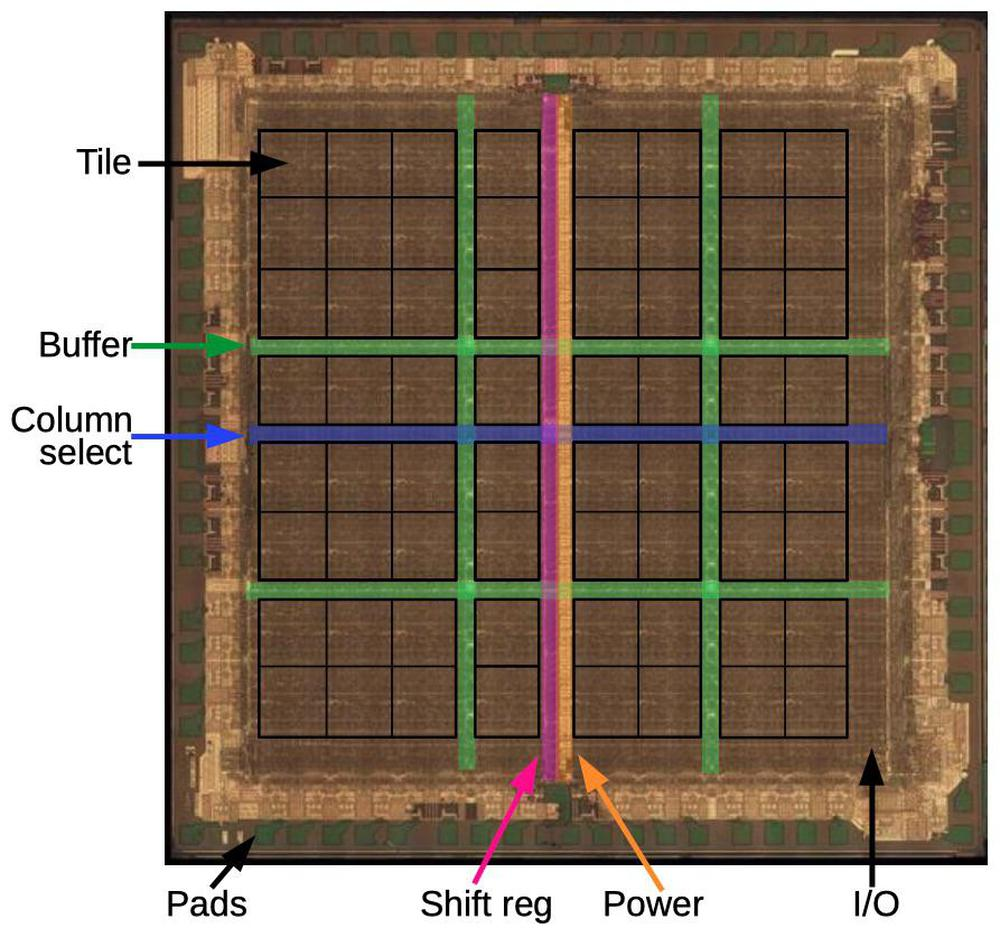Layout of the XC2064 chip. Image from siliconpr0n.

Around the edges of the integrated circuit, I/O blocks provide communication with the outside world. They are connected to the small green square pads, which are wired to the chip's external pins. The die is divided by buffers (green): two vertical and two horizontal. These buffers amplify signals that travel long distances across the circuit, reducing delay. The vertical shift register (pink) and horizontal column select circuit (blue) are used to load the bitstream into the chip, as will be explained below.

Inside a tile

The diagram below shows the layout of a single tile in the XC2064; the chip contains 64 of these tiles packed together as shown above. About 40% of each tile is taken up by the memory cells (green) that hold the configuration bits. The top third (roughly) of the tile handles the interconnect routing through two switch matrices and numerous individual routing switches. Below that is the logic block. Key parts of the logic block are multiplexers for the input, the flip flop, and the lookup tables (LUTs). The tile is connected to neighboring tiles through vertical and horizontal wiring for interconnect, power and ground. The configuration data bits are fed into the memory cells horizontally, while vertical signals select a particular column of memory cells to load.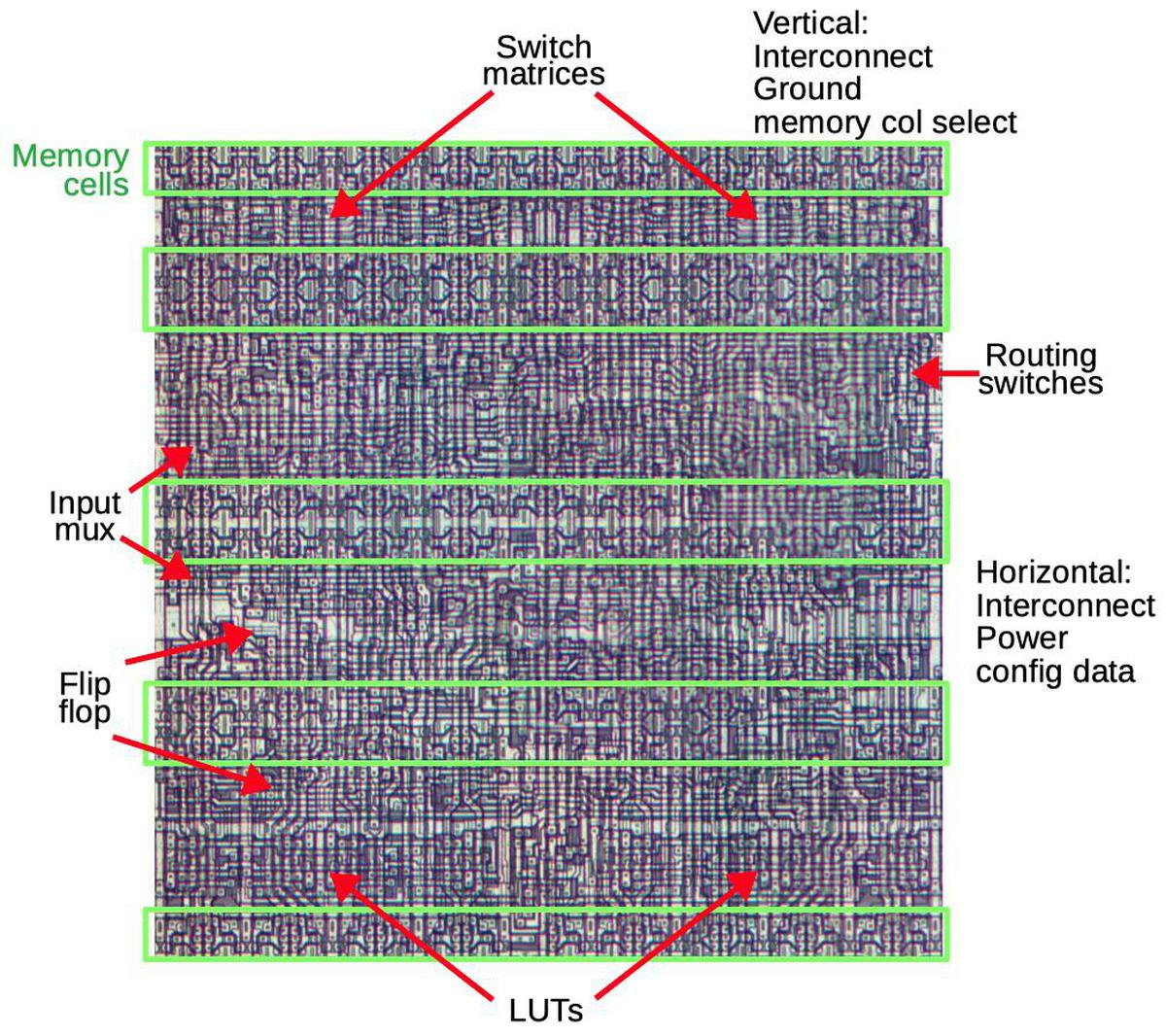One tile of the FPGA, showing important functional units.

Transistors

The FPGA is implemented with CMOS logic, built from NMOS and PMOS transistors. Transistors have two main roles in the FPGA. First, they can be combined to form logic gates. Second, transistors are used as switches that signals pass through, for instance to control routing. In this role, the transistor is called a pass transistor. The diagram below shows the basic structure of an MOS transistor. Two regions of silicon are doped with impurities to form the source and drain regions. In between, the gate turns the transistor on or off, controlling current flow between the source and drain. The gate is made of a special type of silicon called polysilicon, and is separated from the underlying silicon by a thin insulating oxide layer. Above this, two layers of metal provide wiring to connect the circuitry.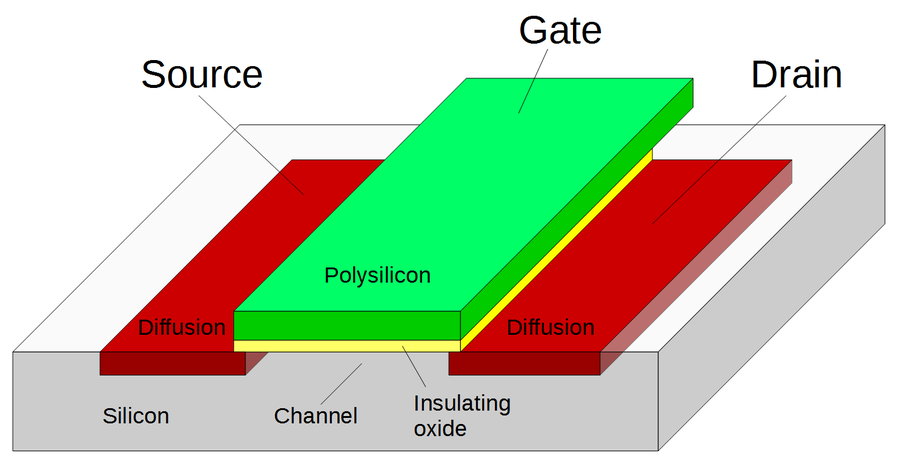Structure of a MOSFET.

The die photo closeup below shows what a transistor looks like under a microscope. The polysilicon gate is the snaking line between the two doped silicon regions. The circles are vias, connections between the silicon and the metal layer (which has been removed for this photo).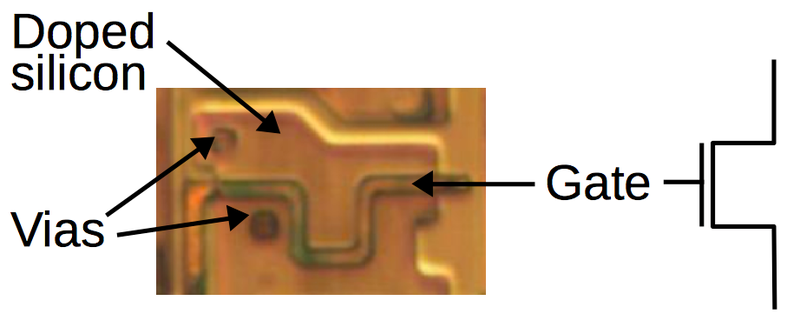A MOSFET as it appears in the FPGA.

The bitstream and configuration memory

The configuration information in the XC2064 is stored in configuration memory cells. Instead of using a block of RAM for storage, the FPGA's memory is distributed across the chip in a 160×71 grid, ensuring that each bit is next to the circuitry that it controls. The diagram below shows how the configuration bitstream is loaded into the FPGA. The bitstream is fed into the shift register that runs down the center of the chip (pink). Once 71 bits have been loaded into the shift register, the column select circuit (blue) selects a particular column of memory and the bits are loaded into this column in parallel. Then, the next 71 bits are loaded into the shift register and the next column to the left becomes the selected column. This process repeats for all 160 columns of the FPGA, loading the entire bitstream into the chip. Using a shift register avoids bulky memory addressing circuitry.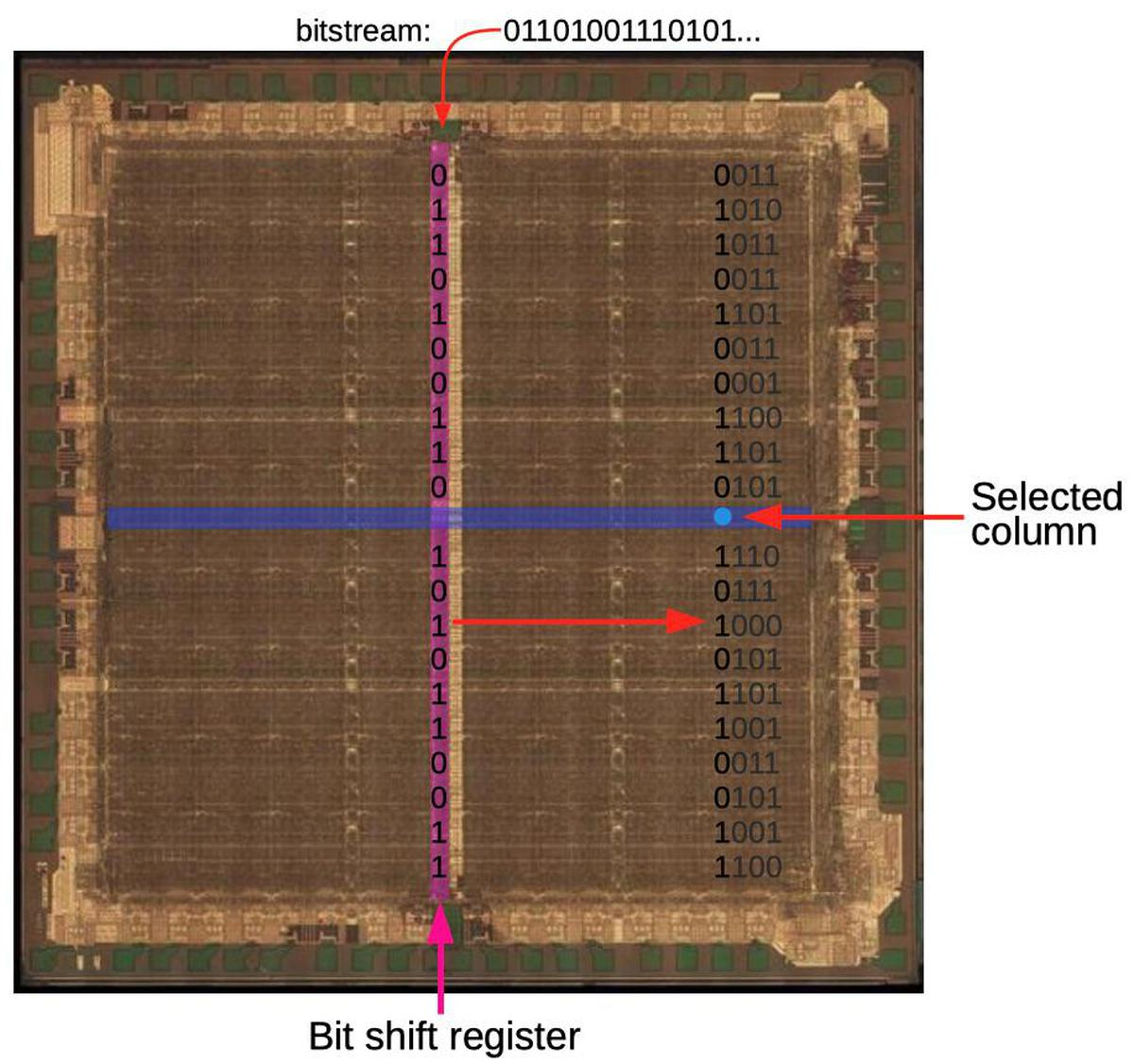How the bitstream is loaded into the FPGA. The bits shown are conceptual; actual bit storage is much denser. The three columns on the left have been loaded and the fourth column is currently being loaded. Die photo from siliconpr0n.

The important point is that the bitstream is distributed across the chip exactly as it appears in the file: the layout of bits in the bitstream file matches the physical layout on the chip. As will be shown below, each bit is stored in the FPGA next to the circuit it controls. Thus, the bitstream file format is directly determined by the layout of the hardware circuits. For instance, when there is a gap between FPGA tiles because of the buffer circuitry, the same gap appears in the bitstream. The content of the bitstream is not designed around software concepts such as fields or data tables or configuration blocks. Understanding the bitstream depends on thinking of it in hardware terms, not in software terms.7

Each bit of configuration memory is implemented as shown below.8 Each memory cell consists of two inverters connected in a loop. This circuit has two stable states so it can store a bit: either the top inverter is 1 and the bottom is 0 or vice versa. To write to the cell, the pass transistor on the left is activated, passing the data signal through. The signal on the data line simply overpowers the inverters, writing the desired bit. (You can also read the configuration data out of the FPGA using the same path.) The Q and inverted Q outputs control the desired function in the FPGA, such as closing a routing connection, providing a bit for a lookup table, or controlling the flip flops. (In most cases, just the Q output is used.)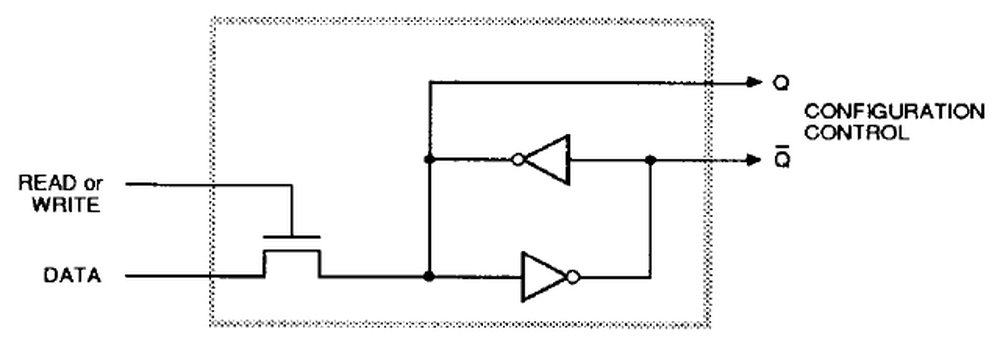Schematic diagram of one bit of configuration memory, from the datasheet. Q is the output and Q is the inverted output.

The diagram below shows the physical layout of memory cells. The photo on the left shows eight memory cells, with one cell highlighted. Each horizontal data line feeds all the memory cells in that row. Each column select line selects all the memory cells in that column for writing. The middle photo zooms in on the silicon and polysilicon transistors for one memory cell. The metal layers were removed to expose the underlying transistors. The metal layers wire together the transistors; the circles are connections (vias) between the silicon or polysilicon and the metal. The schematic shows how the five transistors are connected; the schematic's physical layout matches the photo. Two pairs of transistors form two CMOS inverters, while the pass transistor in the lower left provides access to the cell.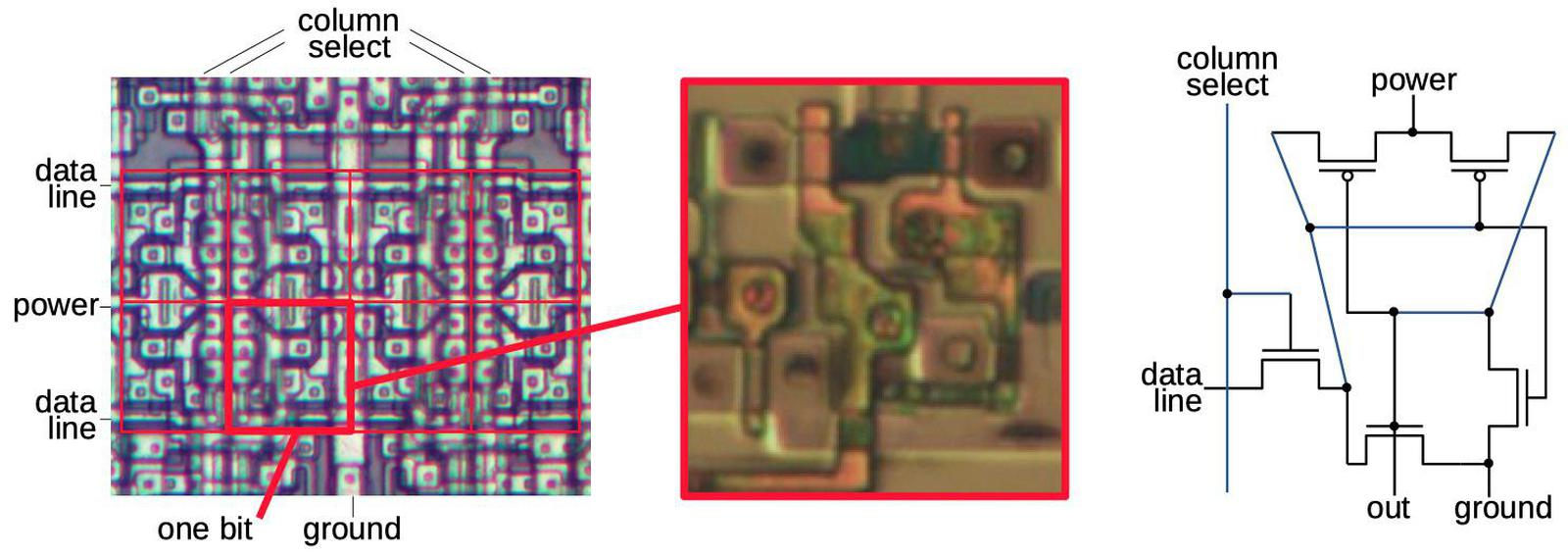Eight bits of configuration memory, four above and four below. The red box shows one bit. When a column select line is activated, the row data line is loaded into the corresponding cells. The closeup and schematic show one bit of configuration memory. Die photo from siliconpr0n.

Lookup table multiplexers

As explained earlier, the FPGA implements arbitrary logic functions by using a lookup table. The diagram below shows how a lookup table is implemented in the XC2064. The eight values on the left are stored in eight memory cells. Four multiplexers select one of each pair of values, depending on the value of the A input; if A is 0, the top value is selected and if A is 1 the bottom value is selected. Next, a larger multiplexer selects one of the four values based on B and C. The result is the desired value, in this case A XOR B XOR C. By putting different values in the lookup table, the logic function can be changed as desired.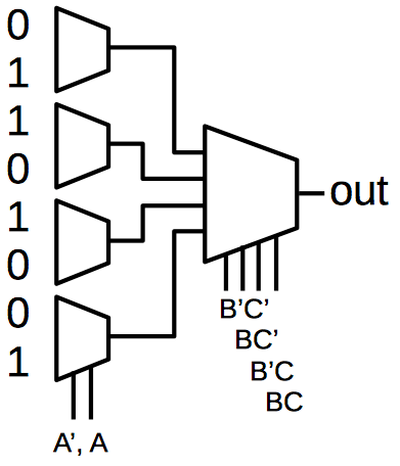Implementing XOR with a lookup table.

Each multiplexer is implemented with pass transistors. Depending on the control signals, one of the pass transistors is activated, passing that input to the output. The diagram below shows part of the LUT circuitry, multiplexing two of the bits. At the right are two of the memory cells. Each bit goes through an inverter to amplify it, and then passes through the multiplexer's pass transistors in the middle, selecting one of the bits.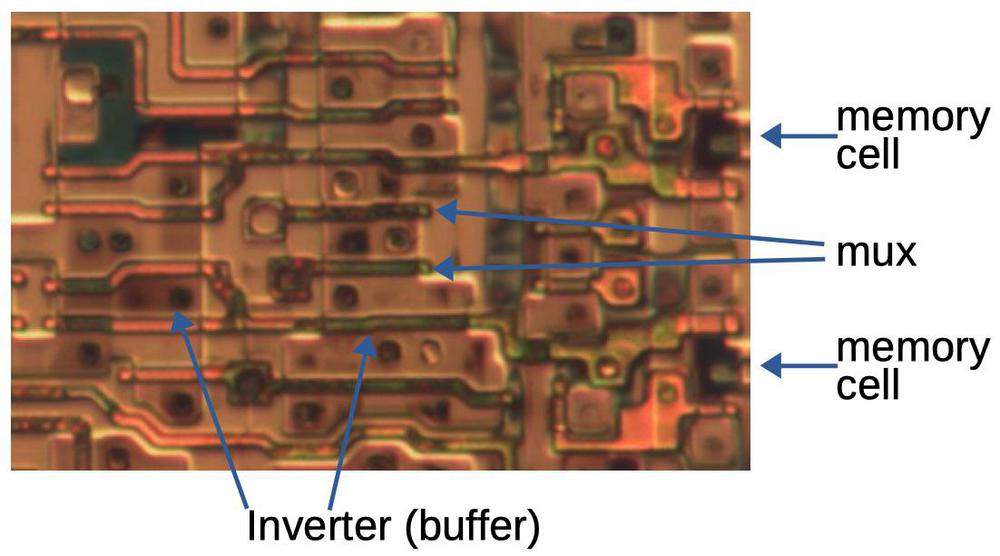A closeup of circuitry in the LUT implementation. Die photo from siliconpr0n.

Flip flop

Each CLB contains a flip flop, allowing the FPGA to implement latches, state machines, and other stateful circuits. The diagram below shows the (slightly unusual) implementation of the flip flop. It uses a primary/secondary design. When the clock is low, the first multiplexer lets the data into the primary latch. When the clock goes high, the multiplexer closes the loop for the first latch, holding the value. (The bit is inverted twice going through the OR gate, NAND gate, and inverter, so it is held constant.) Meanwhile, the secondary latch's multiplexer receives the bit from the first latch when the clock goes high (note that the clock is inverted). This value becomes the flip flop's output. When the clock goes low, the secondary's multiplexer closes the loop, latching the bit. Thus, the flip flop is edge-sensitive, latching the value on the rising edge of the clock. The set and reset lines force the flip flop high or low.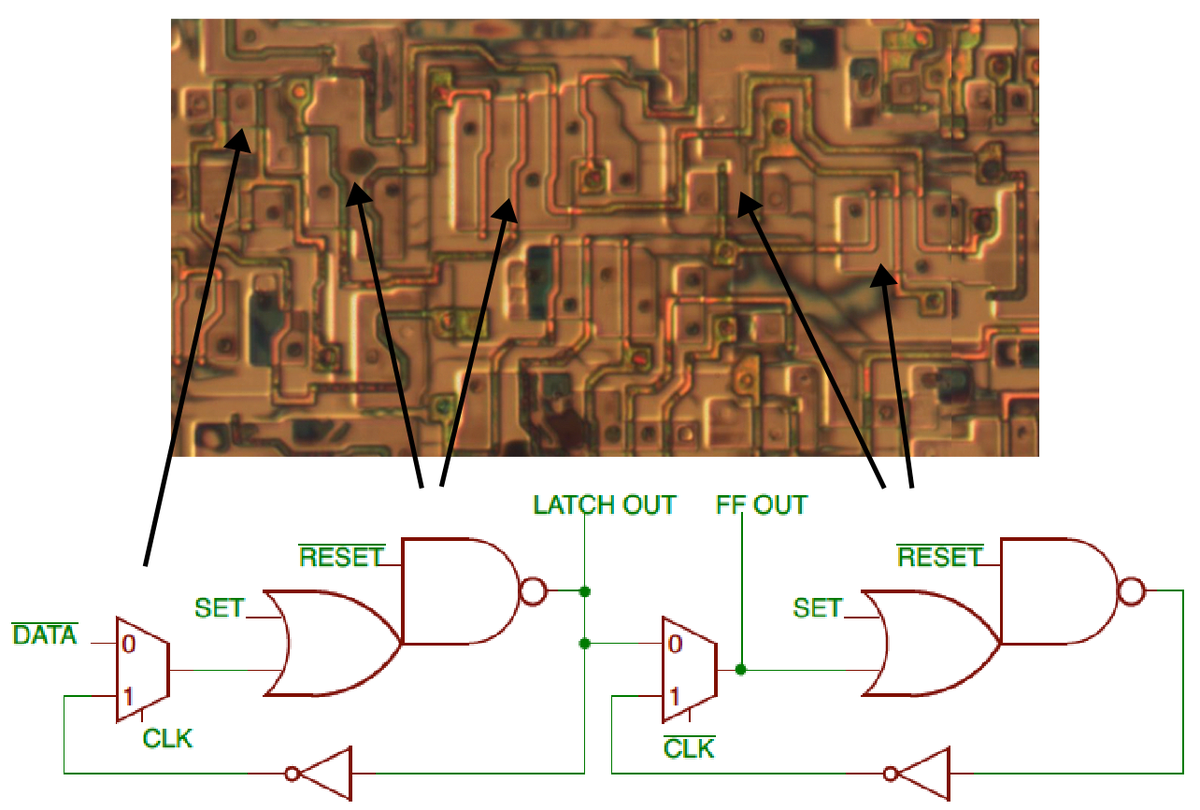Flip flop implementation. The arrows point out the first multiplexer and the two OR-NAND gates. Die photo from siliconpr0n.

8-pin switch matrix

The switch matrix is an important routing element. Each switch has eight "pins" (two on each side) and can connect almost any combination of pins together. This allows signals to turn, split, or cross over with more flexibility than the individual routing nodes. The diagram below shows part of the routing network between four CLBs (cyan). The switch matrices (green) can be connected with any combination of the connections on the right. Note that each pin can connect to 5 of the 7 other pins. For instance, pin 1 can connect to pin 3 but not pin 2 or 4. This makes the matrix almost a crossbar, with 20 potential connections rather than 28.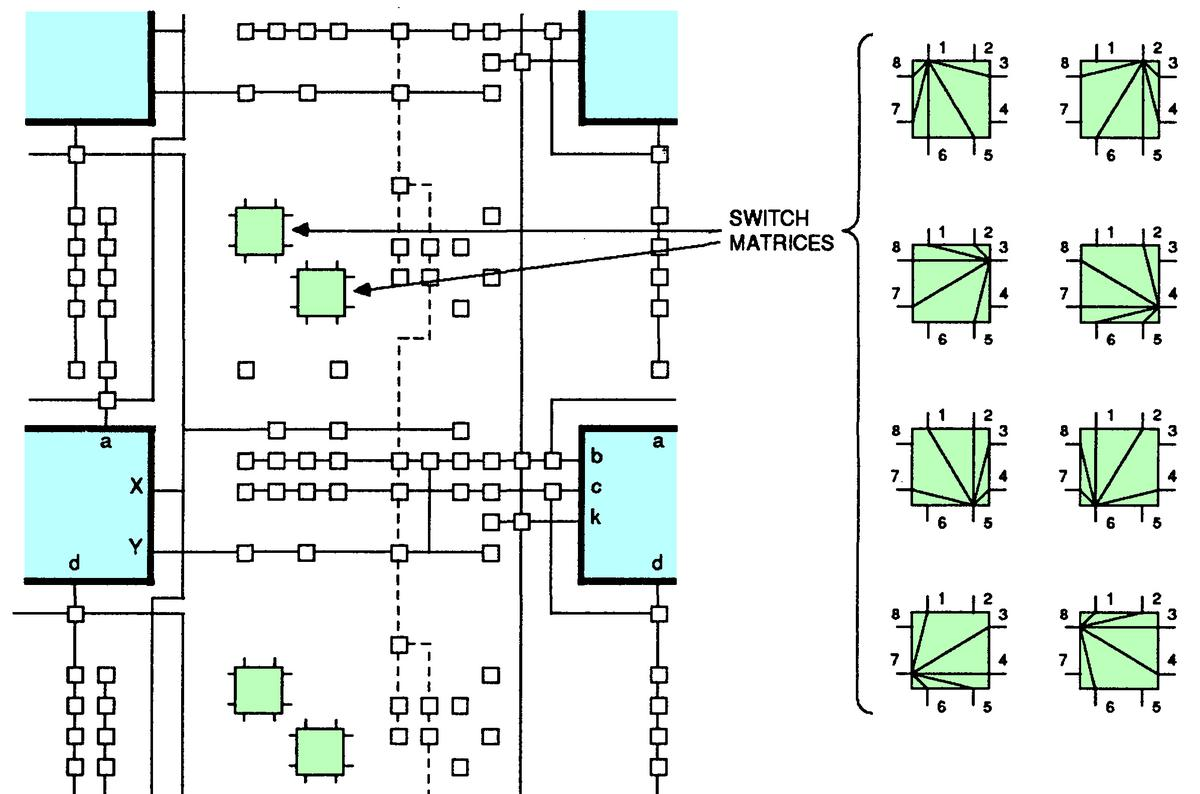The switch matrix is implemented by a row of pass transistors controlled by memory cells above and below. The two sides of the transistor are the two switch matrix pins that can be connected by that transistor. Thus, each switch matrix has 20 associated control bits;9 two matrices per tile yields matrix 40 control bits per tile. The photo below indicates one of the memory cells, connected to the long squiggly gate of the pass transistor below. This transistor controls the connection between pin 5 and pin 1. Thus, the bit in the bitstream corresponding to that memory cell controls the switch connection between pin 5 and pin 1. Likewise, the other memory cells and their associated transistors control other switch connections. Note that the ordering of these connections follows no particular pattern; consequently, the mapping between bitstream bits and the switch pins appears random.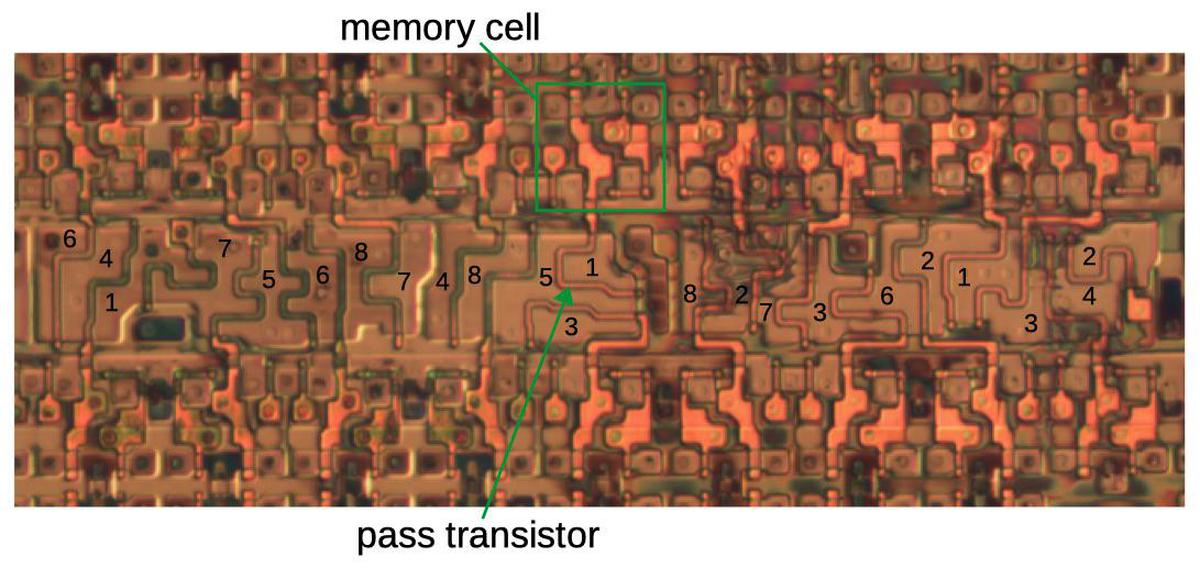Implementation of an 8-pin switch matrix. The silicon regions are labeled with the corresponding pin numbers. The metal layers (which connect the pins to the transistors) were removed for this photo. Based on die photo from siliconpr0n.

Input routing

The inputs to a CLB use a different encoding scheme in the bitstream, which is explained by the hardware implementation. In the diagram below, the eight circled nodes are potential inputs to CLB box DD. Only one node (at most) can be configured as an input, since connecting two signals to the same input would short them together.Input selection. The eight nodes circled in green are potential inputs to DD; one of them can be selected.

The desired input is selected using a multiplexer. A straightforward solution would use an 8-way multiplexer, with 3 control bits selecting one of the 8 signals. Another straightforward solution would be to use 8 pass transistors, each with its own control signal, with one of them selecting the desired signal. However, the FPGA uses a hybrid approach that avoids the decoding hardware of the first approach but uses 5 control signals instead of the eight required by the second approach.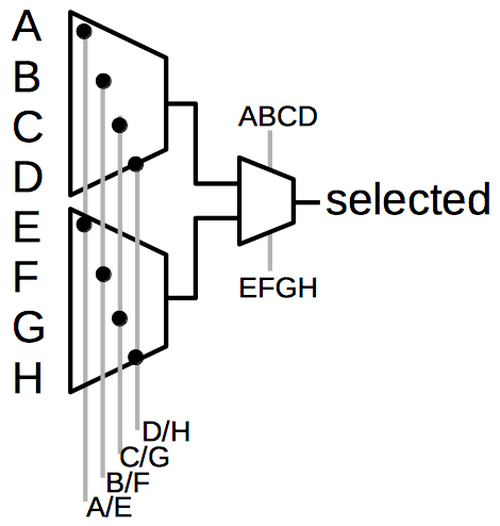The FPGA uses multiplexers to select one of eight inputs.

The schematic above shows the two-stage multiplexer approach used in the FPGA. In the first stage, one of the control signals is activated. The second stage picks either the top or bottom signal for the output.10 For instance, suppose control signal B/F is sent to the first stage and 'ABCD' to the second stage; input B is the only one that will pass through to the output. Thus, selecting one of the eight inputs requires 5 bits in the bitstream and uses 5 memory cells.

Conclusion

The XC2064 uses a variety of highly-optimized circuits to implement its logic blocks and routing. This circuitry required a tight layout in order to fit onto the die. Even so, the XC2064 was a very large chip, larger than microprocessors of the time, so it was difficult to manufacture at first and cost hundreds of dollars. Compared to modern FPGAs, the XC2064 had an absurdly small number of cells, but even so it sparked a revolutionary new product line.

Two concepts are the key to understanding the XC2064's bitstream. First, the FPGA is implemented from 64 tiles, repeated blocks that combine the logic block and routing. Although FPGAs are described as having logic blocks surrounded by routing, that is not how they are implemented. The second concept is that there are no abstractions in the bitstream; it is mapped directly onto the two-dimensional layout of the FPGA. Thus, the bitstream only makes sense if you consider the physical layout of the FPGA.

I've determined how most of the XC2064 bitstream is configured (see footnote 11) and I've made a program to generate the CLB information from a bitstream file. Unfortunately, this is one of those projects where the last 20% takes most of the time, so there's still work to be done. One problem is handling I/O pins, which are full of irregularities and their own routing configuration. Another problem is the tiles around the edges have slightly different configurations. Combining the individual routing points into an overall netlist also requires some tedious graph calculations.

I announce my latest blog posts on Twitter, so follow me at kenshirriff for updates. I also have an RSS feed. Thanks to John McMaster, Tim Ansell and Philip Freidin for discussions.12

Notes and references

1. Ross Freeman tragically died of pneumonia at age 45, five years after inventing the FPGA. In 2009, Freeman was recognized as the inventor of the FPGA by the Inventor's Hall of Fame

2. Xilinx was one of the first fabless semiconductor companies. Unlike most semiconductor companies that designed and manufactured semiconductors, Xilinx only created the design while a fab company did the manufacturing. Xilinx used Seiko Epson Semiconductor Division (as in Seiko watches and Epson printers) for their initial fab.

3. Custom integrated circuits have the problems of high cost and the long time (months or years) to design and manufacture the chip. One solution was Programmable Logic Devices (PLD), chips with gate arrays that can be programmed with various functions, which were developed around 1967. Originally they were mask-programmable; the metal layer of the chip was designed for the desired functionality, a new mask was made, and chips were manufactured to the specifications. Later chips contained a PROM that could be "field programmed" by blowing tiny fuses inside the chip to program it, or an EPROM that could be reprogrammed. Programmable logic devices had a variety of marketing names including Programmable Logic Array, Programmable Array Logic (1978), Generic Array Logic and Uncommitted Logic Array. For the most part, these devices consisted of logic gates arranged as a sum-of-products, although some included flip flops. The main innovation of the FPGA was to provide a programmable interconnect between logic blocks, rather than a fixed gate architecture, as well as logic blocks with flip flops. For an in-depth look at FPGA history and the effects of scalability, see Three Ages of FPGAs: A Retrospective on the First Thirty Years of FPGA Technology. Also see A Brief History of FPGAs

4. The lookup tables in the XC2064 are more complex than just a table. Each CLB contains two 3-input lookup tables. The inputs to the lookup tables in the XC2064 have programmable multiplexers, allowing selection of four different potential inputs. In addition, the two lookup tables can be tied together to create a function on four variables or other combinations.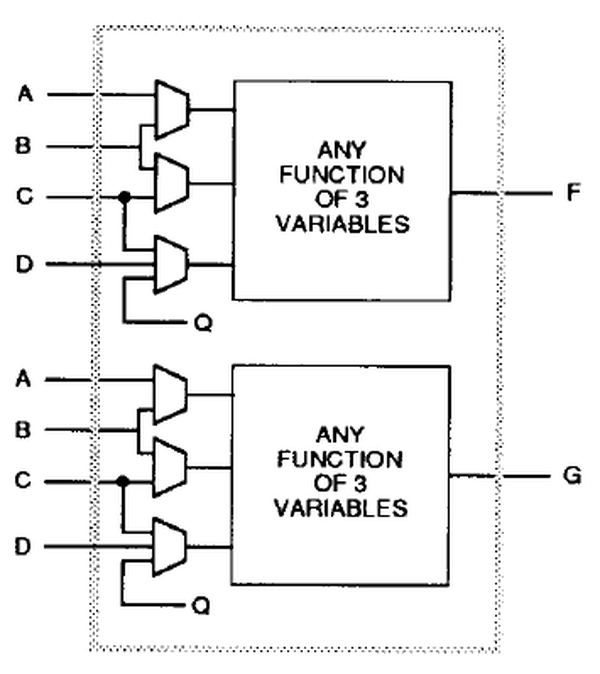Logic functions in the XC2064 FPGA are implemented with lookup tables. From the datasheet.

5. To analyze the XC2064, I used my own die photos of the XC20186 as well as the siliconpr0n photos of the XC2064 and XC2018. Under a light microscope, the FPGA is hard to analyze because it has two metal layers. John McMaster used his electron microscope to help disambiguate the two layers. The photo below shows how the top metal layer is emphasized by the electron microscope.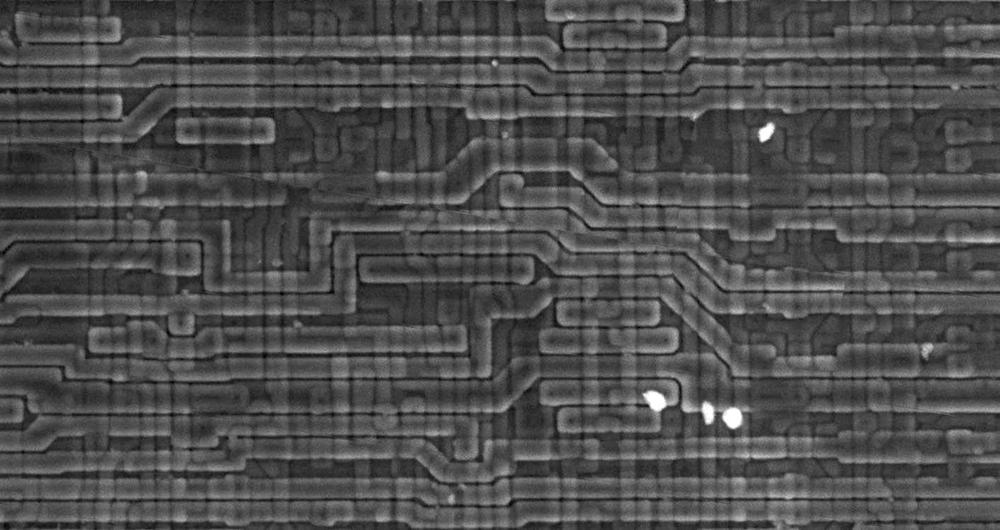Electron microscope photo of the XC2064, courtesy of John McMaster.

6. The Xilinx XC2018 FPGA (below) is a 100-cell version of the XC2064 FPGA. Internally, it uses the same tiles as the 64-cell XC2064, except it has a 10×10 grid of tiles instead of an 8×8 grid. The bitstream format of the XC2018 is very similar, except with more entries.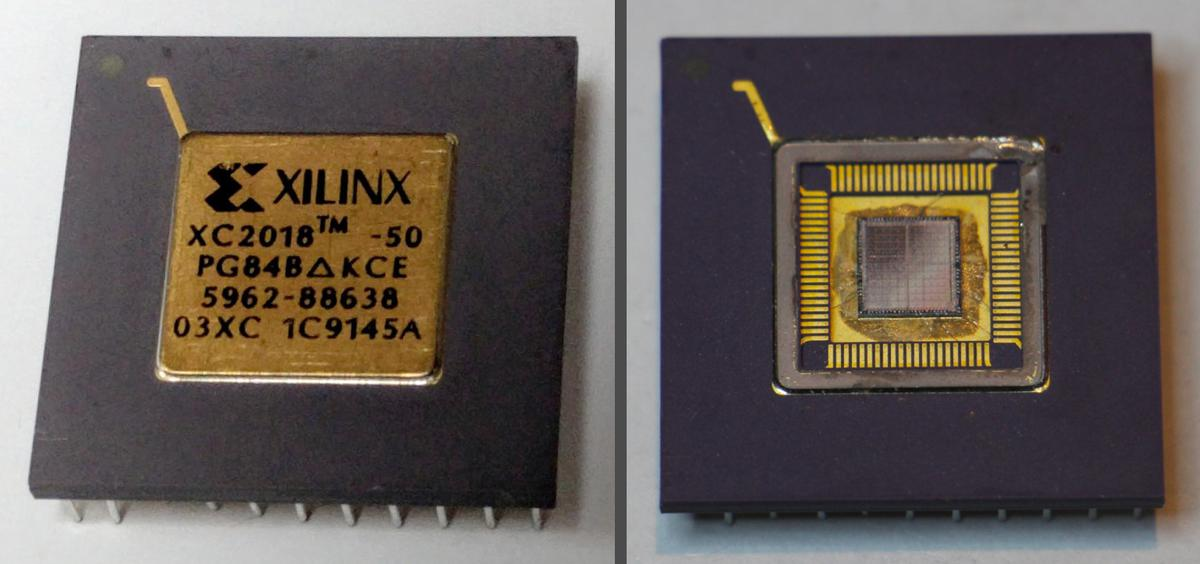The Xilinx XC2018 FPGA. On the right, the lid has been removed, showing the silicon die. The tile pattern is faintly visible on the die.

The image below compares the XC2064 die with the XC2018 die. The dies are very similar, except the larger chip has two more rows and columns of tiles.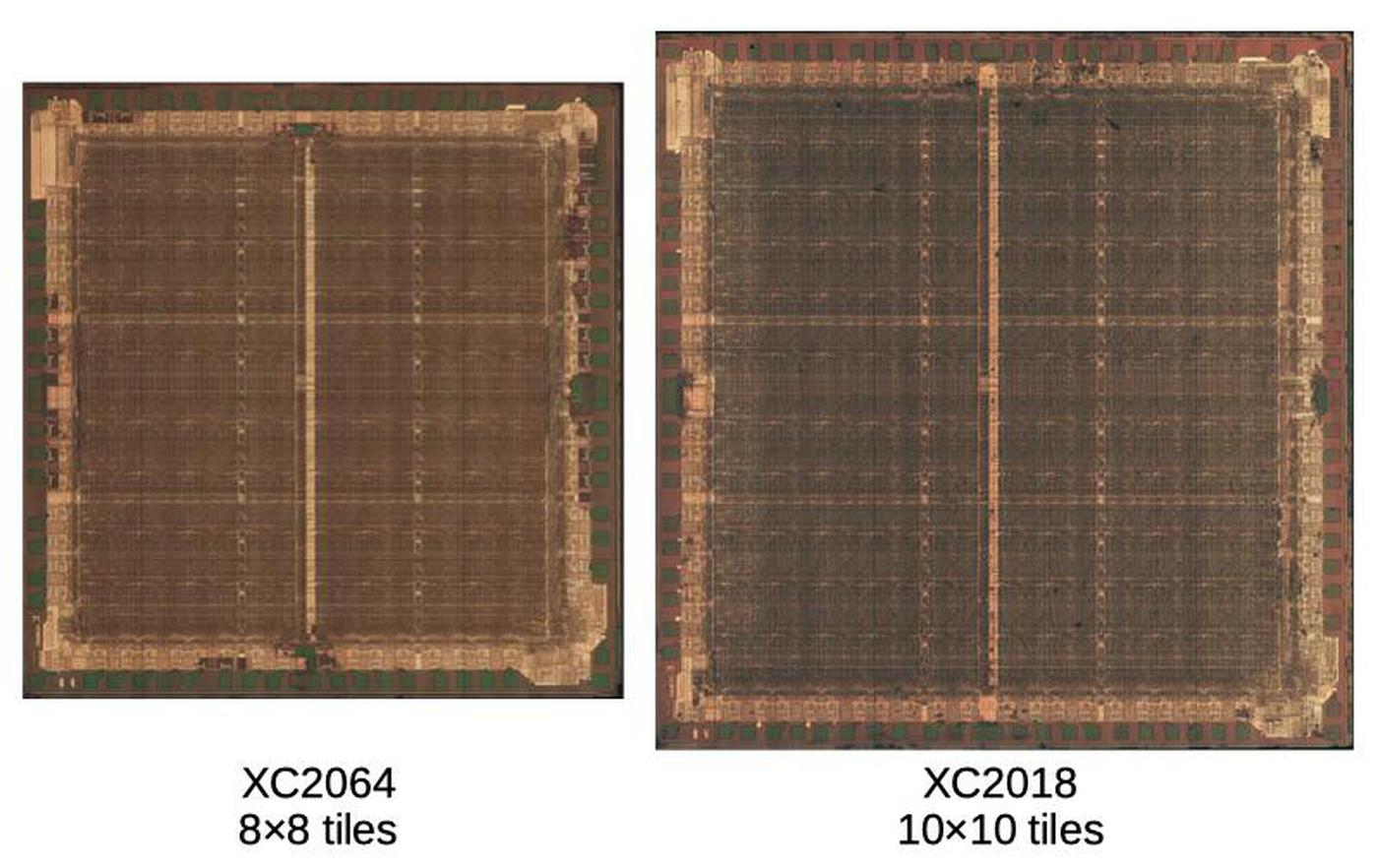Comparison of the XC2064 and XC2018 dies. The images are scaled so the tile sizes match; I don't know how the physical sizes of the dies compare. Die photos from siliconpr0n.

7. While the bitstream directly maps onto the hardware layout, the bitstream file (.RBT) does have a small amount of formatting, shown below.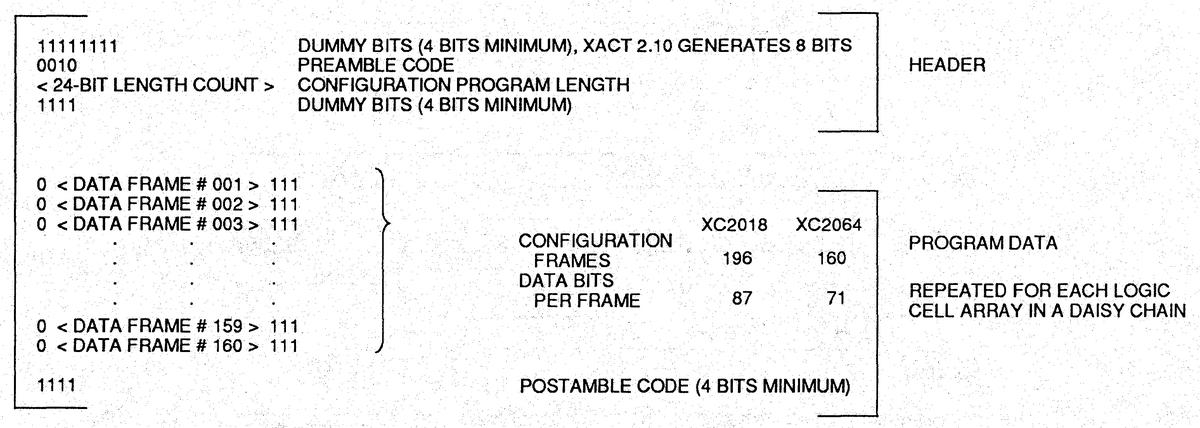The format of the bitstream data, from the datasheet.

8. The configuration memory is implemented as static RAM (SRAM) cells. (Technically, the memory is not RAM since it must be accessed sequentially through the shift register, but people still call it SRAM.) These memory cells have five transistors, so they are known as 5T SRAM.

One question that comes up is if there are any unused bits in the bitstream. It turns out that many bits are unused. For instance, each tile has an 18×8 block of bits assigned to it, of which 27 bits are unused. Looking at the die shows that the memory cell for an unused bit is omitted entirely, allowing that die area to be used for other circuitry. The die photo below shows 9 implemented bits and one missing bit.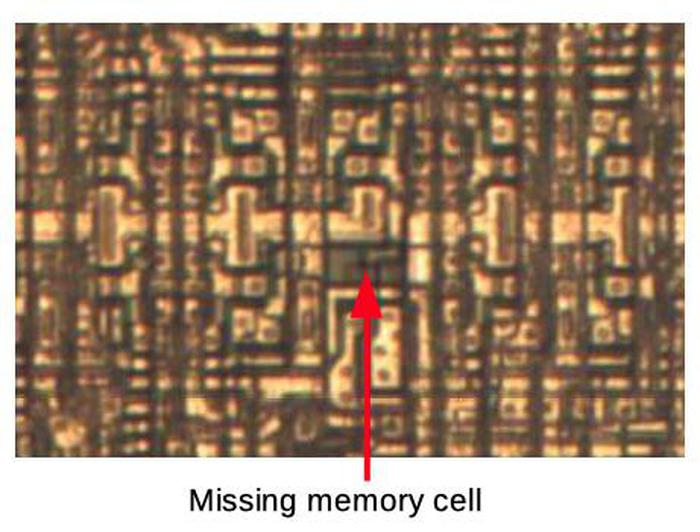Memory cells, showing a gap where one cell is missing. Die photo from siliconpr0n.

9. The switch matrix has 20 pass transistors. Since each tile is 18 memory cells wide, two of the transistors are connected to slightly more distant memory cells.

10. A few notes on the CLB input multiplexer. The control signal EFGH is the complement of ABCD, so only one control signal is needed in the bitstream and only one memory cell for this signal. Second, other inputs to the CLB have 6 or 10 choices; the same two-level multiplexer approach is used, changing the number of inputs and control signals. Finally, a few of the control signals are inverted (probably because the inverted memory output was closer). This can cause confusion when trying to understand the bitstream, since some bits appear to select 6 inputs instead of 2. Looking at the complemented bit, instead, restores the pattern.

11. The following table summarizes the meaning of each bit in a tile's 8×18 part of the bitstream. Each entry in the table corresponds to one bit in the bitstream and indicates what part of the FPGA is controlled by that bit. Empty entries indicate unused bits.

 #2: 1-3 #2: 3-4 PIP D2,D5 (bit inverted) Gin_3 = D G = 1 2' 3' #2: 1-2 #2: 2-6 #2: 2-4 PIP A2,A5 (bit inverted) Gin_3 = C G = 1' 2' 3' #2: 3-7 #2: 3-6 PIP D3, D4, D5 PIP A3, A4, A5 G = 1' 2 3' #2: 2-7 #2: 2-8 ND 11 PIP A1, A4 G = 1 2 3' #2: 1-5 #2: 3-5 PIP A3, AX PIP D1, D4 Y=F G = 1 2' 3 #2: 4-8 #2: 5-8 ND 10 PIP D3, DX Y=G Gin_2 = B G = 1' 2' 3 #2: 7-8 #2: 6-8 ND 9 PIP B2, B5, B6, BX, BY PIP Y2 X=G Gin_1 = A G = 1' 2 3 #2: 5-6 #2: 5-7 ND 8 PIP B3,BX (bit inverted) PIP Y4 X=F G = 1 2 3 #2: 4-6 #2: 1-4 #2: 1-7 PIP C1, C3, C4, C7 PIP X3 Q = LATCH Base FG (separate LUTs) #1: 3-5 #1: 5-8 #1: 2-8 PIP X2 #1: 3-4 #1: 2-4 ND 7 PIP C3,CX (bit inverted) PIP X1 Fin_1 = A F = ! 1 2 3 #1: 1-2 #1: 1-3 ND 6 PIP B6, B7 CLK = enabled Fin_2 = B F = 1' 2 3 #1: 1-5 #1: 1-4 ND 5 PIP C6, C7 CLK = inverted (FF), noninverted (LATCH) F = 1' 2' 3 #1: 4-8 #1: 4-6 ND 4 PIP C4, C5 CLK = C F = 1 2' 3 #1: 2-7 #1: 1-7 ND 3 PIP B4, B5 PIP K1 SET = F F = 1 2 3' #1: 2-6 #1: 3-6 ND 2 PIP B2, BC PIP K2 SET = none F = 1' 2 3' #1: 7-8 #1: 3-7 ND 1 PIP C1, C2 PIP Y3 RES = D or G Fin_3 = C F = 1' 2' 3' #1: 6-8 #1: 5-6 #1: 5-7 PIP B1, BY PIP Y1 RES = G Fin_3 = D F = 1 2' 3'

The first two columns of the table indicate the switch matrices. There are two switch matrices, labeled #1 (red) and #2 (green) in my diagram below. The 8 pins on matrix #1 are labeled 1-8 clockwise. (Switch #2 is the same, but there wasn't room for the labels.) For example, "#2: 1-3" indicates that bit connects pins 1 and 3 on switch #2. The next column defines the "ND" non-directional connections, the boxes below with purple numbers near the switch matrices. Each ND bit in the table controls the corresponding ND connection.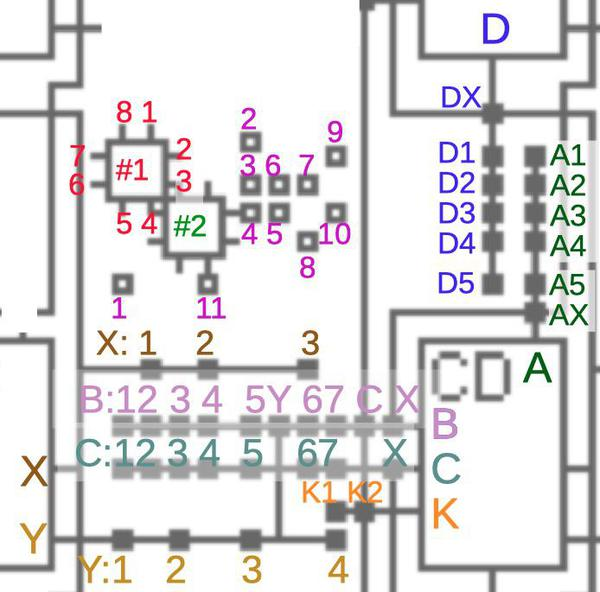Diagram of the interconnect showing the numbering scheme I made up for the bitstream table.

The next two columns describe what I'm calling the PIP connections, the solid boxes on lines above. The connections from output X (brown) are controlled by individual bits (X1, X2, C3). Likewise, the connections from output Y (yellow). The connections to input B (light purple) are different. Only one of these input connections can be active at a time, so they are encoded with multiple bits using the multiplexer scheme. Inputs C (cyan), D (blue) and A (green) are similar. The remaining table columns describe the CLB; refer to the datasheet for details. Bits control the clock, set and reset lines. The X and Y outputs can be selected from the F or G LUTs. The last two columns define the LUTs. There are three inputs for LUT F and three inputs for LUT G, with multiplexers controlling the inputs. Finally, the 8 bits for each LUT are defined, specifying the output for a particular combination of three inputs.

12. Various FPGA patents provide some details on the chips: 4870302, 4642487, 4706216, 4758985, and RE34363. XACT documentation was formerly at Xilinx, but they seem to have removed it. It can now be found here. John McMaster has some xc2064 tools available.